# Dimensionality Reduction Algorithms

Dimensionality reduction is used to remove irrelevant and redundant features.

When the number of features in a dataset is bigger than the number of examples, then the probability density function of the dataset becomes difficult to calculate.

For example, if we model a dataset $$S = \{x^{(i)}\}_{i=1}^m,\ x \in R^{n}$$ as a single Gaussian N(╬╝, Ōłæ), then the probability density function is defined as: $$P(x) = \frac{1}{{(2ŽĆ)}^{\frac{n}{2}} |╬Ż|^\frac{1}{2}} exp(-\frac{1}{2} (x-╬╝)^T {╬Ż}^{-1} (x-╬╝))$$ such as $$╬╝ = \frac{1}{m} \sum_{i=1}^m x^{(i)} \\ Ōłæ = \frac{1}{m} \sum_{i=1}^m (x^{(i)} – ╬╝)(x^{(i)} – ╬╝)^T$$.

But If n >> m, then Ōłæ will be singular, and calculating P(x) will be impossible.

Note: $$(x^{(i)} – ╬╝)(x^{(i)} – ╬╝)^T$$ is always singular, but the $$\sum_{i=1}^m$$ of many singular matrices is most likely invertible when m >> n.

# Principal Component Analysis

Given a set $$S = \{x^{(1)}=(0,1), x^{(2)}=(1,1)\}$$, to reduce the dimensionality of S from 2 to 1, we need to project data on a vector that maximizes the projections. In other words, find the normalized vector $$╬╝ = (╬╝_1, ╬╝_2)$$ that maximizes $$({x^{(1)}}^T.╬╝)^2 + ({x^{(2)}}^T.╬╝)^2 = (╬╝_2)^2 + (╬╝_1 + ╬╝_2)^2$$.

Using the method of Lagrange Multipliers, we can solve the maximization problem with constraint $$||u|| = ╬╝_1^2 + ╬╝_2^2 = 1$$.

$$L(╬╝, ╬╗) = (╬╝_2)^2 + (╬╝_1 + ╬╝_2)^2 – ╬╗ (╬╝_1^2 + ╬╝_2^2 – 1)$$

We need to find ╬╝ such as $$Ōłć_u = 0$$ and ||u|| = 1

After derivations we will find that the solution is the vector ╬╝ = (0.52, 0.85)

Generalization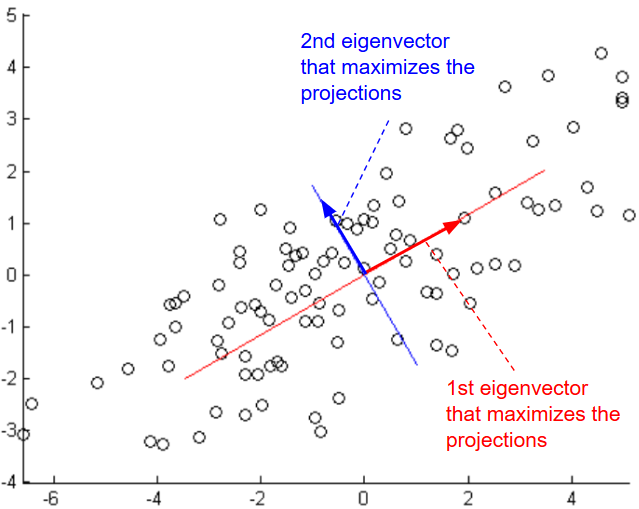Given a set $$S = \{x^{(i)}\}_{i=1}^m,\ x \in R^{n}$$, to reduce the dimensionality of S, we need to find ╬╝ that maximizes $$arg \ \underset{u: ||u|| = 1}{max} \frac{1}{m} \sum_{i=1}^m ({x^{(i)}}^T u)^2$$

$$=\frac{1}{m} \sum_{i=1}^m (u^T {x^{(i)}})({x^{(i)}}^T u)$$ $$=u^T (\frac{1}{m} \sum_{i=1}^m {x^{(i)}} * {x^{(i)}}^T) u$$

LetŌĆÖs define $$Ōłæ = \frac{1}{m} \sum_{i=1}^m {x^{(i)}} * {x^{(i)}}^T$$

Using the method of Lagrange Multipliers, we can solve the maximization problem with constraint $$||u|| = u^Tu$$ = 1.

$$L(╬╝, ╬╗) = u^T Ōłæ u – ╬╗ (u^Tu – 1)$$

If we calculate the derivative with respect to u, we will find:

$$Ōłć_u = Ōłæ u – ╬╗ u = 0$$

Therefore u that solves this maximization problem must be an eigenvector of Ōłæ. We need to choose the eigenvector with highest eigenvalue.

If we choose k eigenvectors $${u_1, u_2, …, u_k}$$, then we need to transform the data by multiplying each example with each eigenvector.

$$x^{(i)} := (u_1^T x^{(i)}, u_2^T x^{(i)},…, , u_k^T x^{(i)}) = U^T x^{(i)}$$

Data should be normalized before running the PCA algorithm:

1-$$╬╝ = \frac{1}{m} \sum_{i=1}^m x^{(i)}$$

2-$$x^{(i)} := x^{(i)} – ╬╝$$

3-$$Žā_j^{(i)} = \frac{1}{m} \sum_{i=1}^m {x_j^{(i)}}^2$$

4-$$x^{(i)} := \frac{x_j^{(i)}}{Žā_j^{(i)}}$$

To reconstruct the original data, we need to calculate $$\widehat{x}^{(i)} := U^T x^{(i)}$$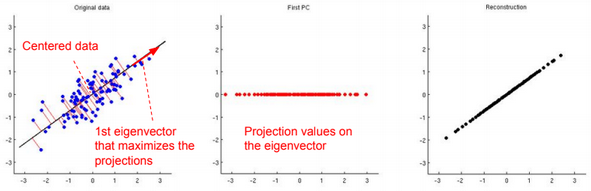# Factor Analysis

Factor analysis is a way to take a mass of data and shrinking it to a smaller data set with less features.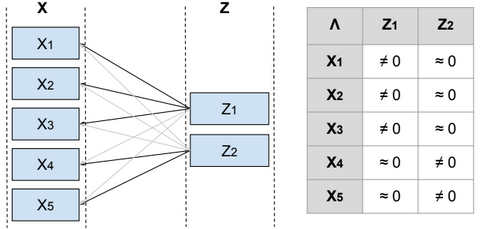Given a set $$S = \{x^{(i)}\}_{i=1}^m,\ x \in R^{n}$$, and S is modeled as a single Gaussian.

To reduce the dimensionality of S, we define a relationship between the variable x and a laten (hidden) variable z called factor such as $$x^{(i)} = ╬╝ + ╬ø z^{(i)} + ŽĄ^{(i)}$$ and $$╬╝ \in R^{n}$$, $$z^{(i)} \in R^{d}$$, $$╬ø \in R^{n*d}$$, $$ŽĄ \sim N(0, ╬©)$$, ╬© is diagonal, $$z \sim N(0, I)$$ and d <= n.

From ╬ø we can find the features that are related to each factor, and then identify the features that need to be eliminated or combined in order to reduce the dimensionality of the data.

Below the steps to estimate the parameters ╬©, ╬╝, ╬ø.

$$E[x] = E[╬╝ + ╬øz + ŽĄ] = E[╬╝] + ╬øE[z] + E[ŽĄ] = ╬╝$$ $$Var(x) = E[(x – ╬╝)^2] = E[(x – ╬╝)(x – ╬╝)^T] = E[(╬øz + ŽĄ)(╬øz + ŽĄ)^T]$$ $$=E[╬øzz^T╬ø^T + ŽĄz^T╬ø^T + ╬øzŽĄ^T + ŽĄŽĄ^T]$$ $$=╬øE[zz^T]╬ø^T + E[ŽĄz^T╬ø^T] + E[╬øzŽĄ^T] + E[ŽĄŽĄ^T]$$ $$=╬ø.Var(z).╬ø^T + E[ŽĄz^T╬ø^T] + E[╬øzŽĄ^T] + Var(ŽĄ)$$

ŽĄ and z are independent, then the join probability of p(ŽĄ,z) = p(ŽĄ)*p(z), and $$E[ŽĄz]=\int_{ŽĄ}\int_{z} ŽĄ*z*p(ŽĄ,z) dŽĄ dz$$

$$=\int_{ŽĄ}\int_{z} ŽĄ*z*p(ŽĄ)*p(z) dŽĄ dz$$ $$=\int_{ŽĄ} ŽĄ*p(ŽĄ) \int_{z} z*p(z) dz dŽĄ$$ $$=E[ŽĄ]E[z]$$

So:

$$Var(x)=╬ø╬ø^T + ╬©$$

Therefore $$x \sim N(╬╝, ╬ø╬ø^T + ╬©)$$ and $$P(x) = \frac{1}{{(2ŽĆ)}^{\frac{n}{2}} |╬ø╬ø^T + ╬©|^\frac{1}{2}} exp(-\frac{1}{2} (x-╬╝)^T {(╬ø╬ø^T + ╬©)}^{-1} (x-╬╝))$$

$$╬ø \in R^{n*d}$$, if d <= m, then $$╬ø╬ø^T + ╬©$$ is most likely invertible.

To find ╬©, ╬╝, ╬ø, we need to maximize the log-likelihood function.

$$l(╬©, ╬╝, ╬ø) = \sum_{i=1}^m log(P(x^{(i)}; ╬©, ╬╝, ╬ø))$$ $$= \sum_{i=1}^m log(\frac{1}{{(2ŽĆ)}^{\frac{n}{2}} |╬ø╬ø^T + ╬©|^\frac{1}{2}} exp(-\frac{1}{2} (x^{(i)}-╬╝)^T {(╬ø╬ø^T + ╬©)}^{-1} (x^{(i)}-╬╝)))$$

This maximization problem cannot be solved by calculating the $$Ōłć_╬© l(╬©, ╬╝, ╬ø) = 0$$, $$Ōłć_╬╝ l(╬©, ╬╝, ╬ø) = 0$$, $$Ōłć_╬ø l(╬©, ╬╝, ╬ø) = 0$$. However using the EM algorithm, we can solve that problem.

More details can be found in this video: https://www.youtube.com/watch?v=ey2PE5xi9-A

# Restricted Boltzmann Machine

A restricted Boltzmann machine (RBM) is a two-layer stochastic neural network where the first layer consists of observed data variables (or visible units), and the second layer consists of latent variables (or hidden units). The visible layer is fully connected to the hidden layer. Both the visible and hidden layers are restricted to have no within-layer connections.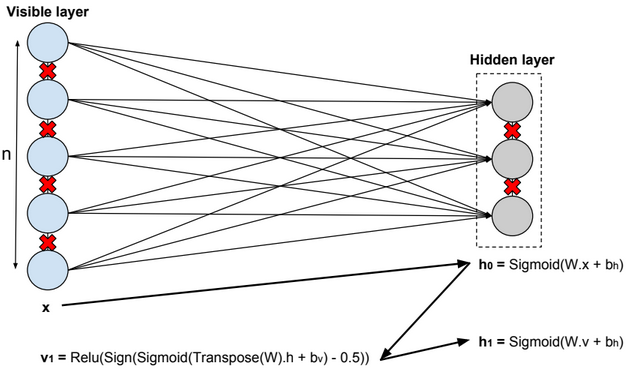In this model, we update the parameters using the following equations:

$$W := W + ╬▒ * \frac{xŌŖŚTranspose(h_0) – v_1 ŌŖŚ Transpose(h_1)}{n} \\ b_v := b_v + ╬▒ * mean(x – v_1) \\ b_h := b_h + ╬▒ * mean(h_0 – h_1) \\ error = mean(square(x – v_1))$$.

# Deep Belief Network

A deep belief network is obtained by stacking several RBMs on top of each other. The hidden layer of the RBM at layer i becomes the input of the RBM at layer i+1. The first layer RBM gets as input the input of the network, and the hidden layer of the last RBM represents the output.

# Autoencoders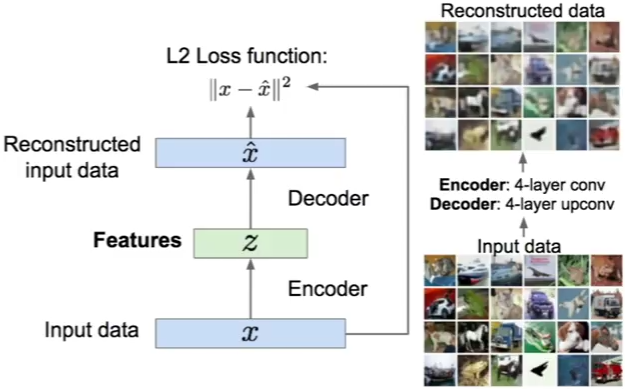An autoencoder, autoassociator or Diabolo network is a deterministic artificial neural network used for unsupervised learning of efficient codings. The aim of an autoencoder is to learn a representation (encoding) for a set of data, typically for the purpose of dimensionality reduction. A deep Autoencoder contains multiple hidden units.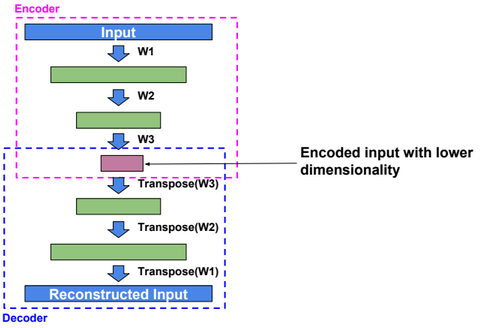Loss function

For binary values, the loss function is defined as:

$$loss(x,\hat{x}) = -\sum_{k=1}^{size(x)} x_k.log(\hat{x_k}) + (1-x_k).log(1 – \hat{x_k})$$.

For real values, the loss function is defined as:

$$loss(x,\hat{x}) = ┬Į \sum_{k=1}^{size(x)} (x_k – \hat{x_k})^2$$.

Dimensionality reduction

Autoencoders separate data better than PCA.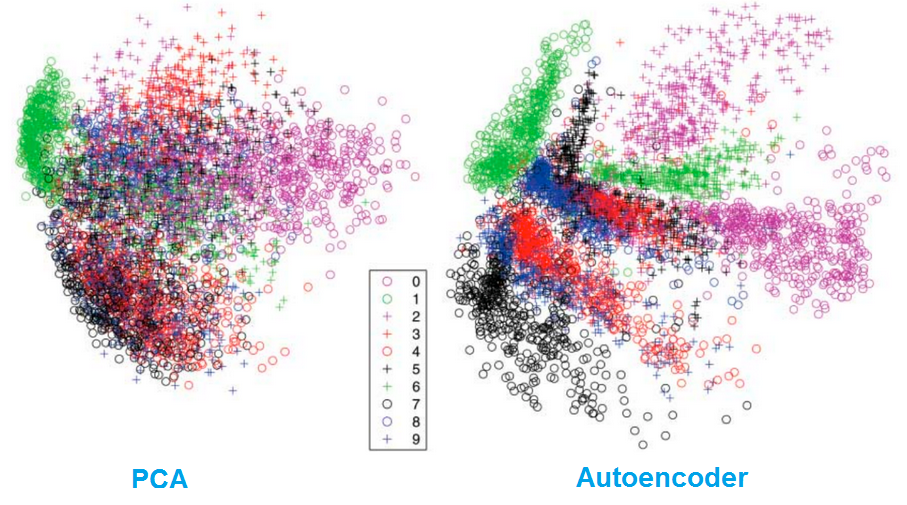# Variational Autoencoder

Variational autoencoder (VAE) models inherit autoencoder architecture, but make strong assumptions concerning the distribution of latent variables. In general, we suppose the distribution of the latent variable is gaussian.

The training algorithm used in VAEs is similar to EM algorithm.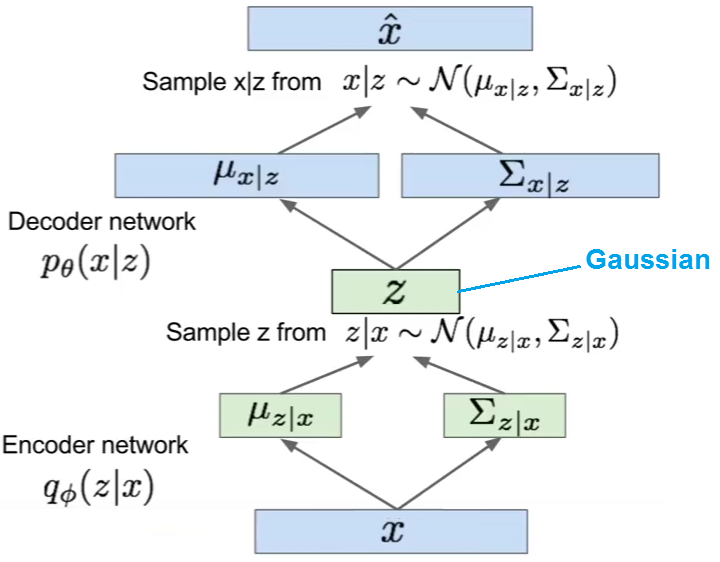# Quantile Regression

Similar to Linear Regression, Quantile Regression estimates the conditional median instead of the conditional mean.

# Decision Tree

Decision Tree is a classification algorithm.

Information Gain

Given the following training set {S}:

 X1 X2 Y a c e a d e b d f b d f

We need to define the first level of the decision tree by choosing one of the two features x1 or x2. We need to calculate the Information Gain (I) for each case, and select the feature with higher Information Gain.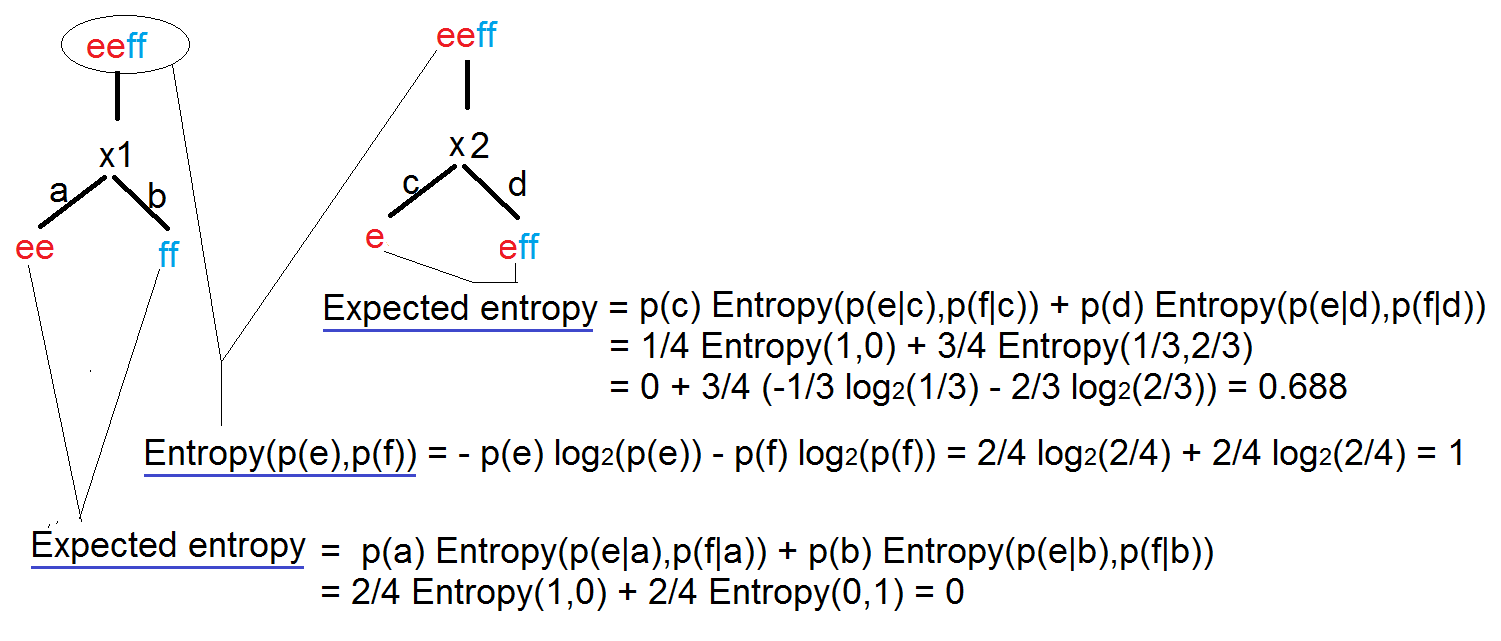$$I(x) = Entropy(parent) – Expected Entropy(children)$$ $$I(X1) = 1 – 0$$ $$I(X2) = 1 – 0.688$$

When training the model, always use data with equal number of rows for each output, so the entropy of the root node equals to 1. (Entropy measure the uncertainty of the data).

Each node of the tree defines a probability estimation for each output.

Standard Deviation Reduction

Another approach to select features that define the levels of a decision tree is by calculating the Standard Deviation Reduction.

# Boosted Decision Tree

A boosted decision tree is an ensemble learning method in which the second tree corrects for the errors of the first tree, the third tree corrects for the errors of the first and second trees, and so forth. The data points which were misclassified receives a bigger weight in the data, the ones correctly classified received a diminished weight. The idea is that with each iteration, the data points misclassified have increasing importance, allowing the learner to fit better and improve accuracy.

Predictions are based on the entire ensemble of trees together that makes the prediction.

# Random Forest

When we have thousand of features (d), calculating the Information Gains for each split point and for each feature requires a lot of time and resources.

The idea in the Random Forest algorithm is to:

1-select a subset of size N from the training data

2-Pick up randomly k features, project the data on the k features, and select the split point with higher information gain.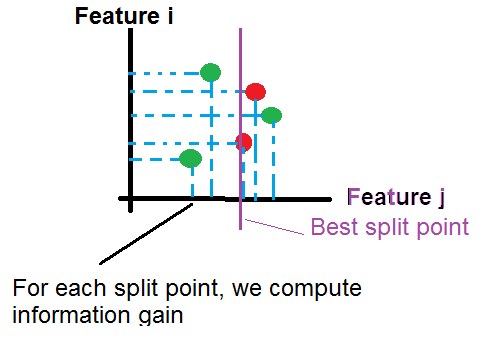Repeat step 2 until the creation of the tree (we can stop the operation at a certain depth of tree).

Repeat step 1 and 2 to generate more trees.

For a new example, we push it through all trees until it reaches the corresponding leaves. then we calculate the probability as the average of probabilities generated by all trees:

$$P(y=k|x) = \frac{1}{nbr\ trees} \sum_{t=1}^{nbr\ trees} P_t(y=k|x)$$

# Clean Missing Data

How estimate missing data?

We can replace missing data with the Mean, the Median or the Mode, but usually it’s not an efficient approach.

If data are multi-variable distributed as below, if there is an example (x0, ?) with a missing value y, we can estimate y as a mean of values y for examples having x near to x0.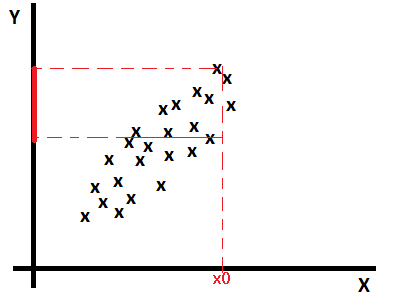Linear regression based imputation

Find a model that estimates missing values based on other features: $$missing\_value = ?_0 + ?_1 * x_1 + ?_2 * x_2 + … + ?_{n+1} * y$$

MICE (Multiple Imputation through Chained Equations)

1-Replace all missing values with random values.

2-Pick randomly a value from one of the three nearest examples. The distance is calculated on values predicted using a regression function(linear, logistic, multinomial). Run the operation for each missing value.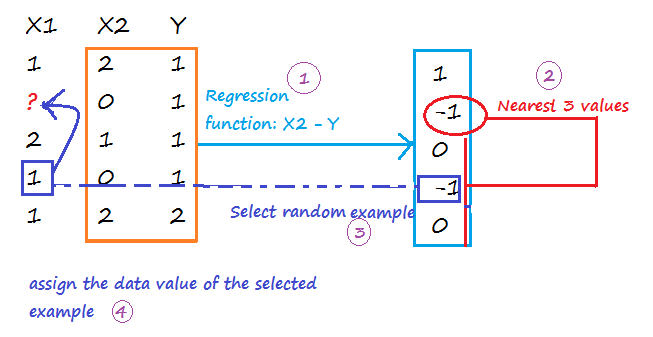Repeat step 1 and 2 m times to create m imputed data sets. Calculate the mean of imputed values from all imputed data sets, and assign it to each missing value of the original data set.

Probabilistic PCA

The PCA approach is based on the calcul of a low-dimensional approximation of the data, from which the full data is reconstructed. The new reconstructed matrix is used to fill missing values.

# Filter based Feature Selection

Pearson Correlation

Pearson correlation coefficient:

$$r_{X,Y} = \frac{Cov(X,Y)}{?_X * ?_Y} \in [-1, 1]$$

Strong positive correlation when $$r_{X,Y}$$ around 1.

No correlation when $$r_{X,Y}$$ around 0.

Strong negative correlation when $$r_{X,Y}$$ around -1.

Mutual Information

$$I(X,Y) = \sum_{x,y} p(x,y)*log(\frac{p(x,y)}{p(x)*p(y)}) >= 0$$

I(X,Y) = 0 when X, Y are independent

Kendall Correlation

Kendall correlation coefficient:

$$? = \frac{C-D}{C+D} \in [-1, 1]$$

C is the number of concordant pairs

D is the number of discordant pairs

 X1 (Sorted) X2 C D 1 2 #values{X2>2 && X1>1}=3 #values{X2<2 && X1>1}=1 2 1 #values{X2>1 && X1>2}=3 #values{X2<1 && X1<2}=0 3 3 #values{X2>3 && X1>3}=2 #values{X2<3 && X1<3}=0 4 5 #values{X2>5 && X1>4}=0 #values{X2<5 && X1<4}=1 5 4 #values{X2>4 && X1>5}=0 #values{X2<4 && X1<5}=0 Total 8 2

$$? = \frac{C-D}{C+D} = 0.6$$ (Good correlation)

Spearman Correlation

Spearman Correlation coefficient:

The Spearman correlation coefficient is defined as the Pearson correlation coefficient between the ranked variables.

$$r_S = \frac{Cov(rg\_X,rg\_Y)}{?_{rg\_X} * ?_{rg\_X}} \in [-1, 1]$$

 X Y rg_X (X rank) rg_Y (Y rank) 1 2 1 2 3 1 2 1 4 4 3 3 6 7 4 4 10 9 5 5
$$r_S = \frac{Cov(rg\_X,rg\_Y)}{?_{rg\_X} * ?_{rg\_Y}} = 0.8$$

Chi-Squared

Example:

 X1 X2 1 2 3 1 3 1 3 1 3 2 3 2
 Occurence X1/X2 1 2 1 0 (3/6) 1 (3/6) 2 0 (0/6) 0 (0/6) 3 3 (15/6) 2 (15/6)

In bold the expected value ((row total*column total)/n).

$$\chi^2 = \frac{(1-3/6)^2}{3/6} + \frac{(0-3/6)^2}{3/6} + \frac{(3-15/6)^2}{15/6} + \frac{(2-15/6)^2}{15/6}=1.2$$

Fisher Score

I don’t know the exact formula used, maybe the coefficient is calculated as below:

$$r = \frac{\sum_{i=1}^{n} (X_i – \overline{X})*(Y_i – \overline{Y})}{\sqrt{(\sum_{i=1}^{n} (X_i – \overline{X})^2) * (\sum_{i=1}^{n} (Y_i – \overline{Y})^2)}}$$

http://www.wikiwand.com/en/Fisher_transformation

Count-Based

Count of values for each feature.

# Skewed Data

When training data are imbalanced (skewed), machine learning algorithms tend to minimize errors for the majority classes on the detriment of minority classes. It usually produces a biased classifier that has a higher predictive accuracy over the majority classes, but poorer predictive accuracy over the minority classes.

Synthetic Minority Oversampling Technique (SMOTE)

SMOTE is an algorithm that creates extra training data by performing certain operations on real data.

Given a set S = {(0,A), (1,A), (2,A), (3,A), (4,B), (5,B)}, A is the majority class, and B the minority class. If the amount of over-sampling needed is 200% (2x) and the number of nearest neighbors is 2, then the algorithm generates new samples in the following way:

Take the difference between an example under consideration and one of its nearest neighbor. Multiply this difference by a random number between 0 and 1, and add it to the feature vector under consideration.

Existing example (4,B) –> New example (4 + 0.3*(4-5), B)

# Model Evaluation

Metrics

 Mean Absolute Error $$\frac{1}{m} \sum_{i=1}^{m} |y – h_?(x)|$$ Root Mean Squared Error (the standard deviation of the distribution of errors) $$\sqrt{\frac{1}{m} \sum_{i=1}^{m} (y – h_?(x))^2} = \sqrt{J(?)}$$ Relative Absolute Error $$\frac{\sum_{i=1}^{m} |y – h_?(x)|}{\sum_{i=1}^{m} |y – \frac{\sum_{i=1}^{m} y}{m}|}$$ Relative Squared Error $$\frac{\sum_{i=1}^{m} (y – h_?(x))^2}{\sum_{i=1}^{m} (y – \frac{\sum_{i=1}^{m} y}{m})^2}$$ Coefficient of Determination ($$R^2$$) 1 – [Relative Squared Error]

True Positive: $$1\{y=1, h_?(x)=1\}$$

False Positive: $$1\{y=0, h_?(x)=1\}$$

True Negative: $$1\{y=0, h_?(x)=0\}$$

False Negative:$$1\{y=1, h_?(x)=0\}$$

 Accuracy $$\frac{[True Positive] + [True Negative]}{m}$$ Precision $$\frac{[True Positive]}{[True Positive] + [False Positive]}$$ Recall $$\frac{[True Positive]}{[True Positive] + [True Negative]}$$ F1 Score $$2 \frac{[Precision]*[Recall]}{[Precision]+[Recall]}$$

ROC Curve

Specificity: True Negative / (True Negative + False Positive)

Sensitivity: True Positive / (True Positive + False Negative)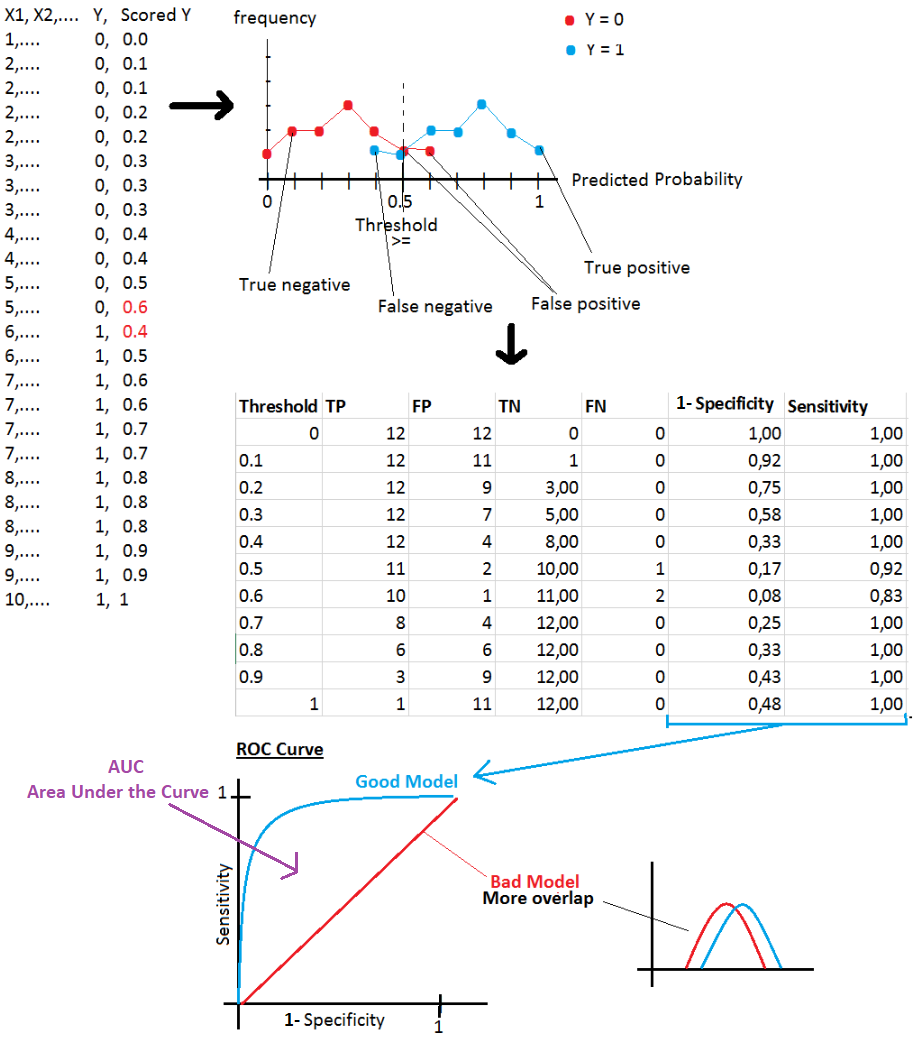# AzureML components

Data Format Conversions

 Convert to Dataset

Data Input/Output

 Enter Data Manually Import Data Import from: Azure Block Storage, Azure SQL Database, Azure Table, Hive Query, Web URL, Data Feed Provider(OData), Azure DocumentDB Export Data

Data Transformation

Rename columns

Join Data

Select Columns in Dataset

Remove Duplicate Rows

Slit Data

Partition and Sample

Select Top xxx rows, or generate a sample, or split data in k folds

Group Data into Bins

Clip Value

Remove outliers (peak and subpeak)

Build Counting Transform

For each selected column, and for an output class, calculate the occurrence of a value in all rows.

Example:

 Country Product Advertised (2 classes) Country Class 0 Count Country Class 1 Count Product Class 0 Count Product Class 1 Count FR P1 0 1 0 2 1 CA P1 0 1 2 2 1 CA P1 1 1 2 2 1 CA P2 1 1 2 0 1

Note: On AzureML, we get wrong results if the number of rows is low.

Statistical Functions

 Summarize Data

Machine Learning

 Train Model Tune Model Hyperparameters Find best hyperparameter values Score Model Evaluate Model Evaluate and compare one or two scored datasets Train Clustering Model

R/Python Language Modules

 Create R Model Execute R Script Execute Python Script Supported Python packages: NumPy, pandas, SciPy, scikit-learn, matplotlib

# Machine Learning Methods

Anomaly Detection Algorithms

 One-Class SVM The algorithm finds a minimal hypersphere around the data. Any point outside the hypersphere is considered an outlier. PCA-Based Anomaly Detection The algorithm applies PCA to reduce the dimensionality of the data, and applying distance metrics to identify cases that represent anomalies.

Classification Algorithms

 Logistic Regression Neural Network Averaged Perceptron Averaged Perceptron algorithm is an extension of the standard Perceptron algorithm; it uses averaged weights and biases. Bayes Point Machine ItÆs Bayesian linear classifier, therefore the model is not prone to overfitting Decision Forest Decision Jungle Boosted Decision Tree Decision Forest Decision Jungle SVM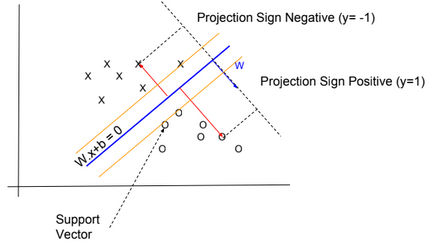The algorithm finds an optimal hyperplane that categorizes new examples. I didnÆt know which kernel function is used by the algorithm. Probably it uses a linear kernel $$K(x,xÆ) = x^T .xÆ)$$ SVM is not recommended with large training sets, because the classification of new examples requires the computation of kernels using all training examples. Locally Deep SVM The algorithm defines a tree to cluster examples (like Decision Tree algorithm), and then trains SVM on each cluster. One-vs-All Multiclass The One-vs-All Multiclass requires as an input multiple two-class classification algorithms. To classify a new example, the algorithm runs two-class classification algorithms for each class, then select the class with higher probability.

Clustering Algorithms

 K-Means Clustering

Regression Algorithms

 Bayesian Linear Regression This model is not prone to overfitting Boosted Decision Tree Regression Decision Forest Regression Fast Forest Quantile Regression Neural Network Regression Neural network with an output layer having no logistic function Linear Regression Ordinal Regression Algorithm used for predicting an ordinal variable. Poisson Regression Algorithm used for predicting a Poisson variable

# Machine Learning Algorithms – Part 3

This post describes some geometric machine learning algorithms.

# K-Nearest Neighbor Regression

k-nearest neighbors regression algorithm (k-NN regression) is a non-parametric method used for regression. For a new example x, predict y as the average of values $$y_1, y_2, …, y_k$$ of k nearest neighbors of x.

# K-Nearest Neighbor Classifier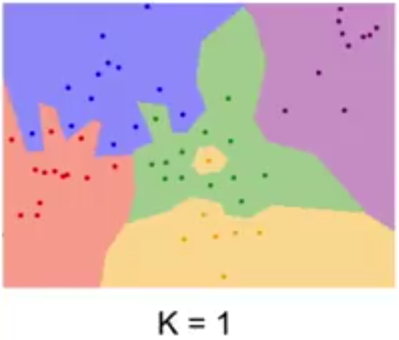k-nearest neighbors classifier (k-NN classifier) is a non-parametric method used for classification. For a new example x, predict y as the most common class among the k nearest neighbors of x.

# Support Vector Machine (SVM)In SVM, y has two values -1 or 1, and the hypothesis function is $$h_{W,b}(x) = g(W^T x + b)$$ with g(z) = {1 if z >= 0, otherwise -1}.

We need to find W and b so $$W^T x + b$$ >> 0 when y=1 and $$W^T x + b$$ << 0 when y=-1

Functional margin

$$\widehat{?}^{(i)} = y^{(i)} . (W^T x^{(i)} +b)$$

We want that this function be >> 0 for all values of x.

We define $$\widehat{?} = min \ \widehat{?}^{(i)}$$ for all values of i

Geometric margin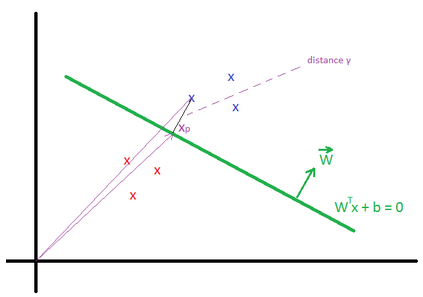Geometric margin $$?^{(i)}$$ is the geometric distance between an example $$x^{(i)}$$ and the line defined by $$W^Tx + b = 0$$. We define $$x_p^{(i)}$$ as the point of projection of $$x^{(i)}$$ on the line.

The vector $$\overrightarrow{x}^{(i)} = \overrightarrow{x_p}^{(i)} + ?^{(i)} * \frac{\overrightarrow{W}}{||W||}$$

$$x_p^{(i)}$$ is in the line, then $$W^T (x^{(i)} – ?^{(i)} * \frac{W}{||W||}) + b = 0$$

We can find that $$?^{(i)} = \frac{W^T x^{(i)} + b}{||W||}$$

More generally $$?^{(i)} = y^{(i)}(\frac{W^T x^{(i)}}{||W||} + \frac{b}{||W||})$$

We define $$? = min {?}^{(i)}$$ for all values of i

We can deduce that the Geometric margin(?) = Functional margin / ||W||

Max Margin Classifier

We need to maximize the geometric margin $$?$$, in other words $$\underset{W, b, ?}{max}$$ ?, such as $$y^{(i)}(\frac{W^T x^{(i)}}{||W||} + \frac{b}{||W||})$$ >= ? for all values $$(x^{(i)},y^{(i)})$$.

To simplify the maximization problem, we impose a scaling constraint: $$\widehat{?} = ? * ||W|| = 1$$.

The optimization problem becomes as follow: $$\underset{W, b}{max}\frac{1}{||W||}$$ (or $$\underset{W, b}{min}\frac{1}{2}||W||^2$$), such as $$y^{(i)}(W^T x^{(i)} + b)$$ >= 1 for all values $$(x^{(i)},y^{(j)})$$.

Using the method of Lagrange Multipliers, we can solve the minimization problem.

$$L(W, b, ?) = \frac{1}{2} ||W||^2 – \sum_{i=1}^m ?_i * (y^{(i)}(W^T x^{(i)} + b) – 1)$$

Based on the KKT conditions, $$?_i >= 0$$ and $$?_i * (y^{(i)}(W^T x^{(i)} + b) – 1) = 0$$ for all i.

We know that $$y^{(i)}(W^T x^{(i)} + b) – 1 = 0$$ is true only for few examples (called support vectors) located close to the the line $$W^Tx + b = 0$$ (for these examples, $$? = {?}^{(i)} = \frac{1}{||W||}$$), therefore $$?_i$$ is equal to 0 for the majority of examples.

By deriving L, we can find:

$$\frac{\partial L(W, b, ?)}{\partial w} = W – \sum_{i=1}^m ?_i * y^{(i)} * x^{(i)} = 0 \\ \frac{\partial L(W, b, ?)}{\partial b} = \sum_{i=1}^m ?_i * y^{(i)} = 0$$

After multiple derivations, we can find the equations for W, b and ?.

More details can be found in this video: https://www.youtube.com/watch?v=s8B4A5ubw6c

Predictions

The hypothesis function is defined as: $$h_{W,b}(x) = g(W^T x + b) = g(\sum_{i=1}^m ?_i * y^{(i)} * K(x^{(i)}, x) + b)$$

The function $$K(x^{(i)}, x) = ?(x^{(i)})^T ?(x)$$ is known as the kernel function (? is a mapping function).

When ?(x) = x, then $$h_{W,b}(x) = g(\sum_{i=1}^m ?_i * y^{(i)} * (x^{(i)})^T . x + b)$$

Kernels

There are many types of kernels: ōPolynomial kernelö, ōGaussian kernelö,…

In the example below the kernel is defined as Quadratic Kernel. $$K(x^{(i)}, x) = ?(x^{(i)})^T ?(x)$$ and $$?(x) = (x_1^2, x_2^2, \sqrt{2} x_1 x_2)$$. By using this kernel, SVM can find a linear separator (or hyperplane).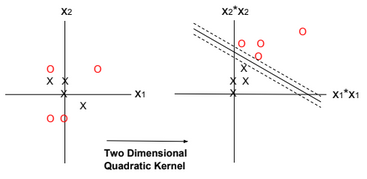Regularization

Regularization can be applied to SVM by adding an extra term to the minimization function. The extra term relaxes the constraints by introducing slack variables. C is the regularization parameter.

$$\underset{W, b, ?_i}{min}\frac{||W||^2}{2} \color{red} {+ C \sum_{i=1}^m ?_i }$$, such as $$y^{(i)}(W^T x^{(i)} + b)$$ >= 1 – ?i for all values $$(x^{(i)},y^{(j)})$$ and $$?_i >= 0$$.

The regularization parameter serves as a degree of importance that is given to miss-classifications. SVM poses a quadratic optimization problem that looks for maximizing the margin between both classes and minimizing the amount of miss-classifications. However, for non-separable problems, in order to find a solution, the miss-classification constraint must be relaxed.

As C grows larger, wrongly classified examples are not allowed, and when C tends to zero the more miss-classifications are allowed.

# One-Class SVM

One-Class SVM is used to detect outliers. It finds a minimal hypersphere around the data. In other words, we need to minimize the radius R.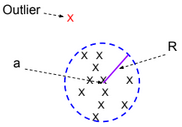The minimization function for this model is defined as follow:

$$\underset{R, a, ?_i}{min} ||R||^2 + C \sum_{i=1}^m ?_i$$, such as $$||x^{(i)} – a||^2 \leq R^2 + ?i$$ for all values $$(x^{(i)},y^{(j)})$$ and $$?_i >= 0$$.

# Perceptron Algorithm

Similar to SVM, the Perceptron algorithm tries to separate positive and negative examples. There is no notion of kernel or margin maximization when using the perceptron algorithm. The algorithm can be trained online.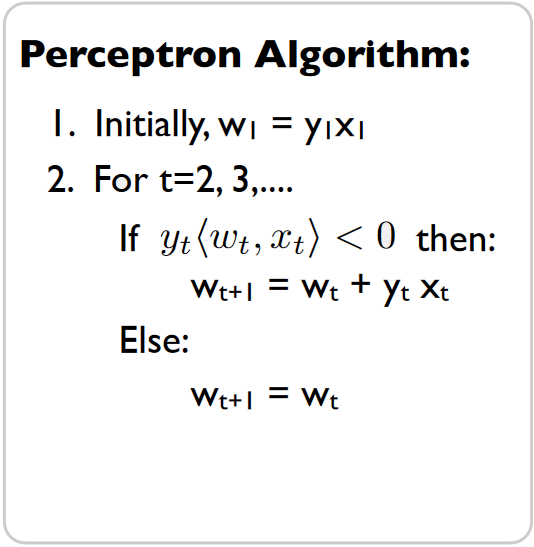# Averaged Perceptron Algorithm

Averaged Perceptron Algorithm is an extension of the standard Perceptron algorithm; it uses averaged weights and biases.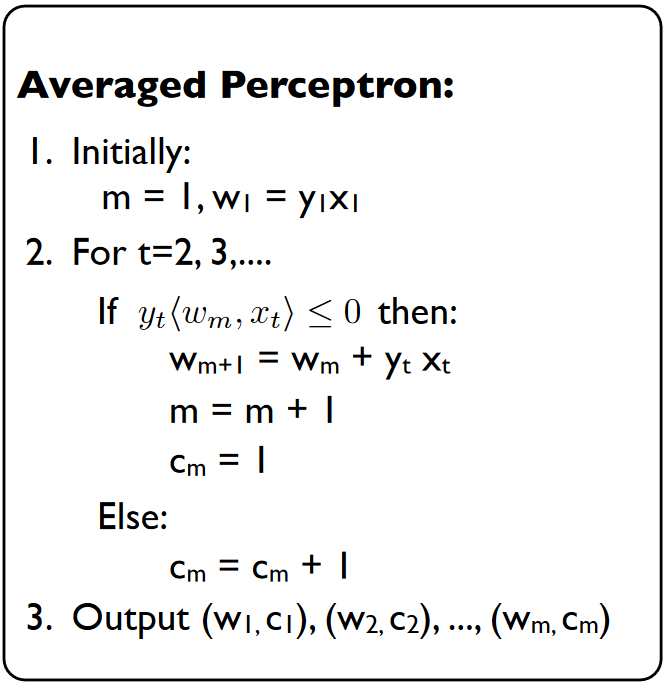The classification of a new example is calculated by evaluating the following expression:

$$? = sign(\sum_{i=1}^m c_i.w_i^T.x + b_i)$$

Note: the sign of wx+b is related to the location of the point x.

w.x+b = w(x1+x2)+b = w.x2 because x1 is located on the blue line then w.x1+b = 0.

w.x2 is positive if the two vectors have the same direction.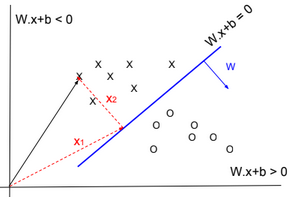# K-means

Given a training set $$S = \{x^{(i)}\}_{i=1}^m$$. We define k as the number of clusters (groups) to create. Each cluster has a centroid $$\mu_k$$.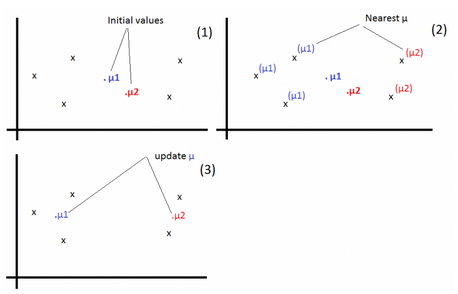1-For each example, find j that minimizes: $$c^{(i)} := arg\ \underset{j}{min} ||x^{(i)} – \mu_j||^2$$

2-Update $$\mu_j:=\frac{\sum_{i=1}^m 1\{c^{(i)} = j\} x^{(i)}}{\sum_{i=1}^m 1\{c^{(i)} = j\}}$$

Repeat the operations 1 & 2 until convergence.

# Large/Small Neural Networks

For a large amount of training data, use large networks.

# Applying Machine Learning

Below the steps to apply machine learning to a business problem.

1-Collect DataSet

2-Prepare DataSet

3-Select & Design Model

4-Train Model

5-Evaluate Model

6-Test Model

# Train/Dev/Test Sets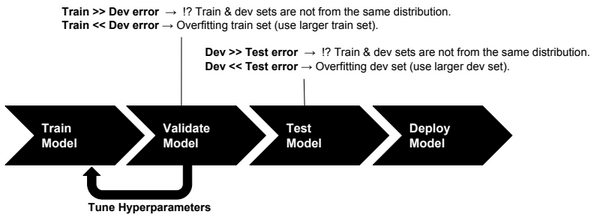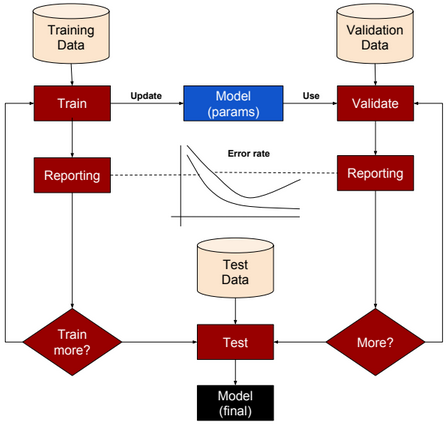Training set: a set of examples used for training a ML model.

Dev (Validation or Cross-Validation) set: a set of examples used to tune hyper-parameters of a ML model.

Test set: a set of examples used to evaluate how well the model does with data outside the training/Dev set.

For deep learning algorithms, we no longer need to follow the common rules of (70%, 30%) ratios for Train and Test sets or (70%, 15%, 15%) ratios for Train, Dev and Test sets. Splitting data into 98% as training set and 2% as test set could be an acceptable option.

# Error Analysis

Let us define first the following acronyms:

TP: True positive

FP: False positive

TN: True negative

FN: False negative

 Accuracy (TP + TN) / (TP + TN + FP + FN) Precision TP / (TP + FP) Recall TP / (TP + FN) F1 score (best error metric) 2 (Precision * Recall) /(Precision + Recall)

Accuracy alone is a bad measure when data is skewed.

# Machine Learning Algorithms – Part 2

This post describes some generative machine learning algorithms.

# Gaussian Discriminant Analysis

Gaussian Discriminant Analysis is a supervised classification algorithm. In this algorithm we suppose the p(x|y) is multivariate Gaussian with parameter mean $$\overrightarrow{u}$$ and covariance $$\Sigma : P(x|y) \sim N(\overrightarrow{u}, \Sigma)$$.

If we suppose that y is Bernoulli distributed, then there are two distributions that can be defined, one for p(x|y=0) and one for p(x|y=1). The separator between these two distributions could be used to predict the values of y.

$$P(x|y=0) = \frac{1}{(2\pi)^{\frac{n}{2}}|\Sigma|^{\frac{1}{2}}} exp(-\frac{1}{2}(x – \mu_0)^T\Sigma^{-1}(x – \mu_0))$$

$$P(x|y=1) = \frac{1}{(2\pi)^{\frac{n}{2}}|\Sigma|^{\frac{1}{2}}} exp(-\frac{1}{2}(x – \mu_1)^T\Sigma^{-1}(x – \mu_1))$$

$$P(y) = \phi^y.(1-\phi)^{1-y}$$

We need to find $$\phi, \mu_0, \mu_1, \Sigma$$ that maximize the log-likelihood function:

$$l(\phi, \mu_0, \mu_1, \Sigma) = log(\prod_{i=1}^{m} P(y^{(i)}|x^{(i)};\phi, \mu_0, \mu_1, \Sigma))$$

Using the Naive Bayes rule, we can transform the equation to:

$$= log(\prod_{i=1}^{m} P(x^{(i)} | y^{(i)};\mu_0, \mu_1, \Sigma) * P(y^{(i)};\phi))) – log(\prod_{i=1}^{m} P(x^{(i)}))$$

To maximize l, we need to maximize: $$log(\prod_{i=1}^{m} P(x^{(i)} | y^{(i)};\mu_0, \mu_1, \Sigma) * P(y^{(i)};\phi)))$$

For new data, to predict y, we need to calculate $$P(y=1|x) = \frac{P(x|y=1) * P(y=1)}{P(x|y=0)P(y=0) + P(x|y=1)P(y=1)}$$ (P(y=0) and P(y=1) can be easily calculated from training data).

# EM Algorithm

EM algorithm is an unsupervised clustering algorithm in which we assign a label to each training example. The clustering applied is soft clustering, which means that for each point we assign a probability for each label.

For a point x, we calculate the probability for each label.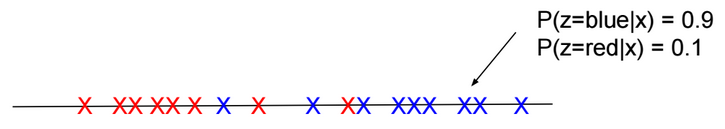There are two main steps in this algorithm:

E-Step: Using the current estimate of parameters, calculate if points are more likely to come from the red distribution or the blue distribution.

M-Step: Re-estimate the parameters.

Given a training set $$S = \{x^{(i)}\}_{i=1}^m$$. For each $$x^{(i)}$$, there is hidden label $$z^{(i)}$$ assigned to it.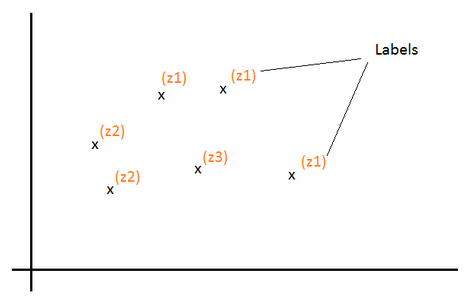Z is a multinomial distribution with $$P(z = j)=?_j$$.

k the number of distinct values of Z (number of classes).

For each $$x^{(i)}$$, we would like to know the value $$z^{(i)}$$ that is assigned to it. In other words, find $$l \in [1, k]$$ that maximizes the probability $$P(z^{(i)} = l | x^{(i)})$$

Log-likelihood function to maximize: $$l(?) = log(\prod_{i=1}^m P(x^{(i)}; ?)) = \sum_{i=1}^m log(P(x^{(i)}; ?))$$

$$= \sum_{i=1}^m log(\sum_{l=1}^k P(x^{(i)}|z^{(i)} = l; ?) * P(z^{(i)} = l))$$ $$= \sum_{i=1}^m log(\sum_{l=1}^k P(x^{(i)}, z^{(i)} = l; ?))$$

Let’s define $$Q_i$$ as a probability distribution over Z: ($$Q_i(z^{(i)} = l)$$ > = 0 and $$\sum_{l=1}^k Q_i(z^{(i)} = l) = 1$$)

$$l(?) = \sum_{i=1}^m log(\sum_{l=1}^k Q_i(z^{(i)} = l) \frac{P(x^{(i)}, z^{(i)} = l; ?)}{Q_i(z^{(i)} = l)})$$ $$= \sum_{i=1}^m log(E_{z^{(i)} \sim Q_i}[\frac{P(x^{(i)}, z^{(i)}; ?)}{Q_i(z^{(i)})}])$$

Log is concave function. Based on Jensen’s inequality log(E[X]) >= E[log(X)].

Then:

$$l(?) >= \sum_{i=1}^m E_{z^{(i)} \sim Q_i}[log(\frac{P(x^{(i)}, z^{(i)}; ?)}{Q_i(z^{(i)})})]$$ $$= \sum_{i=1}^m \sum_{l=1}^k Q_i(z^{(i)}=l) log(\frac{P(x^{(i)}, z^{(i)}=l; ?)}{Q_i(z^{(i)}=l)})$$

If we set $$Q_i(z^{(i)}) = P(z^{(i)}| x^{(i)}; ?)$$ then: $$\frac{P(x^{(i)}, z^{(i)}; ?)}{Q(z^{(i)})}$$ $$= \frac{P(z^{(i)}| x^{(i)}; ?) * P(x^{(i)}; ?)}{P(z^{(i)}| x^{(i)}; ?)}$$ $$= P(x^{(i)}; ?)$$ = Constant (over z). In that case log(E[X]) = E[log(X)] and for the current estimate of ?, $$l(?) = \sum_{i=1}^m \sum_{l=1}^k Q_i(z^{(i)}=l) log(\frac{P(x^{(i)}, z^{(i)}=l; ?)}{Q_i(z^{(i)}=l)})$$ (E-Step)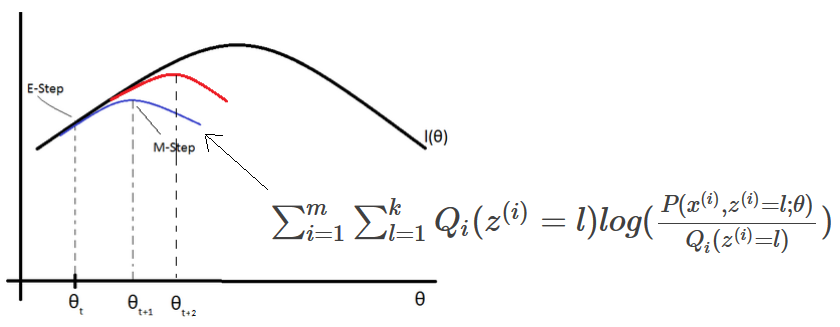EM (Expectation-Maximization) algorithm for density estimation:

1-Initiliaze the parameters ?

2-For each i, set $$Q_i(z^{(i)}) = P(z^{(i)}| x^{(i)}; ?)$$ (E-Step)

3-Update ? (M-Step)

$$? := arg\ \underset{?}{max} \sum_{i=1}^m \sum_{l=1}^k Q_i(z^{(i)}=l) log(\frac{P(x^{(i)}, z^{(i)}=l; ?)}{Q_i(z^{(i)}=l)})$$

Mixture of Gaussians

Given a value $$z^{(i)}=j$$, If x is distributed normally $$N(?_j, ?_j)$$, then:

$$Q_i(z^{(i)} = j) = P(z^{(i)} = j| x^{(i)}; ?)$$ $$= \frac{P(x^{(i)} | z^{(i)}=j) * P(z^{(i)} = j)}{\sum_{l=1}^k P(x^{(i)} | z^{(i)}=l) * P(z^{(i)}=l)}$$ $$= \frac{\frac{1}{{(2?)}^{\frac{n}{2}} |?_j|^\frac{1}{2}} exp(-\frac{1}{2} (x^{(i)}-?_j)^T {?_j}^{-1} (x^{(i)}-?_j)) * ?_j}{\sum_{l=1}^k \frac{1}{{(2?)}^{\frac{n}{2}} |?_l|^\frac{1}{2}} exp(-\frac{1}{2} (x^{(i)}-?_l)^T {?_l}^{-1} (x^{(i)}-?_l)) * ?_l}$$

Using the method of Lagrange Multipliers, we can find $$?_j, ?_j, ?_j$$ that maximize: $$\sum_{i=1}^m \sum_{l=1}^k Q_i(z^{(i)}=l) log(\frac{\frac{1}{{(2?)}^{\frac{n}{2}} |?_j|^\frac{1}{2}} exp(-\frac{1}{2} (x^{(i)}-?_j)^T {?_j}^{-1} (x^{(i)}-?_j)) * ?_j}{Q_i(z^{(i)}=l)})$$ with the constraint $$\sum_{j=1}^k ?_j = 1$$. We also need to set to zero the partial derivatives with respect to $$?_j$$ and $$?_j$$ and solve the equations to find the new values of these parameters. Repeat the operations until convergence.

# Naive Bayes Classifier

Naive Bayes classifier is a supervised classification algorithm. In this algorithm, we try to calculate P(y|x), but this probability can be calculated using the naive bayes rule:

P(y|x) = P(x|y).P(y)/P(x)

If x is the vector $$[x_1,x_2,…,x_n]$$, then:

$$P(x|y) = P(x_1,x_2,…,x_n|y) = P(x_1|y) P(x_2|y, x_1) ….P(x_n|y, x_{n-1},…,x_1)$$ $$P(x) = P(x1,x2,…,x_n)$$

If we suppose that $$x_i$$ are conditionally independent (Naive Bayes assumption), then:

$$P(x|y) = P(x_1|y) * P(x_2|y) * … * P(x_n|y)$$ $$= \prod_{i=1}^n P(x_i|y)$$ $$P(x) = \prod_{i=1}^n P(x_i)$$

If $$\exists$$ i such as $$P(x_i|y)=0$$, then P(x|y) = 0. To avoid that case we need to use Laplace Smoothing when calculating probabilities.

We suppose that $$x_i \in \{0,1\}$$ (one-hot encoding: [1,0,1,…,0]) and y $$\in \{0,1\}$$.

We define $$\phi_{i|y=1}, \phi_{i|y=0}, \phi_y$$ such as:

$$P(x_i=1|y=1) = \phi_{i|y=1} \\ P(x_i=0|y=1) = 1 – \phi_{i|y=1}$$ $$P(x_i=1|y=0) = \phi_{i|y=0} \\ P(x_i=0|y=0) = 1 – \phi_{i|y=0}$$ $$P(y) = \phi_y^y.(1-\phi_y)^{1-y}$$

We need to find $$\phi_y, \phi_{i|y=0}, \phi_{i|y=1}$$ that maximize the log-likelihood function:

$$l(\phi, \phi_{i|y=0}, \phi_{i|y=1}) = log(\prod_{i=1}^{m} \prod_{j=1}^n P(x_j^{(i)}|y^{(i)}) P(y^{(i)})/P(x^{(i)}))$$

Or maximize the following expression:

$$log(\prod_{i=1}^{m} \prod_{j=1}^n P(x_j^{(i)}|y^{(i)}) P(y^{(i)}))$$

# Machine Learning Algorithms – Part 1

This post describes some discriminative machine learning algorithms.

 Distribution of Y given X Algorithm to predict Y Normal distribution Linear regression Bernoulli distribution Logistic regression Multinomial distribution Multinomial logistic regression (Softmax regression) Exponential family distribution Generalized linear regression
 Distribution of X Algorithm to predict Y Multivariate normal distribution Gaussian discriminant analysis or EM Algorithm X Features conditionally independent $$p(x_1, x_2|y)=p(x_1|y) * p(x_2|y)$$ Naive Bayes Algorithm

Other ML algorithms are based on geometry, like the SVM and K-means algorithms.

# Linear Regression

Below a table listing house prices by size.

 x = House Size ($$m^2$$) y = House Price (k\$) 50 99 50 100 50 100 50 101 60 110

For the size 50$$m^2$$, if we suppose that prices are normally distributed around the mean ╬╝=100 with a standard deviation Žā, then P(y|x = 50) = $$\frac{1}{\sigma \sqrt{2\pi}} exp(-\frac{1}{2} (\frac{y-╬╝}{\sigma})^{2})$$

We define h(x) as a function that returns the mean of the distribution of y given x (E[y|x]). We will define this function as a linear function. $$E[y|x] = h_{╬Ė}(x) = \theta^T x$$

P(y|x = 50; ╬Ė) = $$\frac{1}{\sigma \sqrt{2\pi}} exp(-\frac{1}{2} (\frac{y-h_{╬Ė}(x)}{\sigma})^{2})$$

We need to find ╬Ė that maximizes the probability for all values of x. In other words, we need to find ╬Ė that maximizes the likelihood function L:

$$L(\theta)=P(\overrightarrow{y}|X;╬Ė)=\prod_{i=1}^{n} P(y^{(i)}|x^{(i)};╬Ė)$$

Or maximizes the log likelihood function l:

$$l(\theta)=log(L(\theta )) = \sum_{i=1}^{m} log(P(y^{(i)}|x^{(i)};\theta ))$$ $$= \sum_{i=1}^{m} log(\frac{1}{\sigma \sqrt{2\pi}}) -\frac{1}{2} \sum_{i=1}^{n} (\frac{y^{(i)}-h_{╬Ė}(x^{(i)})}{\sigma})^{2}$$

To maximize l, we need to minimize J(╬Ė) = $$\frac{1}{2} \sum_{i=1}^{m} (y^{(i)}-h_{╬Ė}(x^{(i)}))^{2}$$. This function is called the Cost function (or Energy function, or Loss function, or Objective function) of a linear regression model. It’s also called “Least-squares cost function”.

J(╬Ė) is convex, to minimize it, we need to solve the equation $$\frac{\partial J(╬Ė)}{\partial ╬Ė} = 0$$. A convex function has no local minimum.

There are many methods to solve this equation:

• Normal equation
• Newton method
• Matrix differentiation

Gradient descent is the most used Optimizer (also called Learner or Solver) for learning model weights.

$$╬Ė_{j} := ╬Ė_{j} – \alpha \frac{\partial J(╬Ė)}{\partial ╬Ė_{j}} = ╬Ė_{j} – ╬▒ \frac{\partial \frac{1}{2} \sum_{i=1}^{n} (y^{(i)}-h_{╬Ė}(x^{(i)}))^{2}}{\partial ╬Ė_{j}}$$

╬▒ is called “Learning rate”

$$╬Ė_{j} := ╬Ė_{j} – ╬▒ \frac{1}{2} \sum_{i=1}^{n} \frac{\partial (y^{(i)}-h_{╬Ė}(x^{(i)}))^{2}}{\partial ╬Ė_{j}}$$

If $$h_{╬Ė}(x)$$ is a linear function ($$h_{╬Ė} = ╬Ė^{T}x$$), then :

$$╬Ė_{j} := ╬Ė_{j} – ╬▒ \sum_{i=1}^{n} (h_{╬Ė}(x^{(i)}) – y^{(i)}) * x_j^{(i)}$$

Batch size should fit the size of CPU or GPU memories, otherwise learning speed will be extremely slow.

When using Batch gradient descent, the cost function in general decreases without oscillations.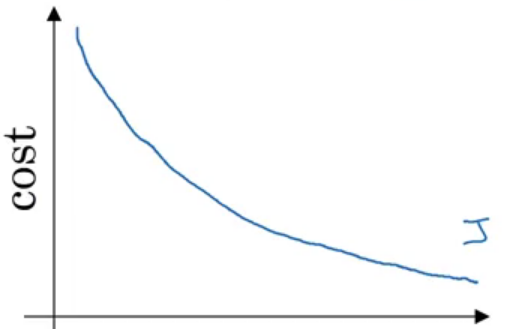Stochastic (Online) Gradient Descent (SGD) (use one example for each iteration – pass through all data N times (N Epoch))

$$╬Ė_{j} := ╬Ė_{j} – \alpha (h_{╬Ė}(x^{(i)}) – y^{(i)}) * x_j^{(i)}$$

This learning rule is called “Least mean squares (LMS)” learning rule. It’s also called Widrow-Hoff learning rule.

Run gradient descent for each mini-batch until we pass through traning set (1 epoch). Repeat the operation many times.

$$╬Ė_{j} := ╬Ė_{j} – \alpha \sum_{i=1}^{20} (h_{╬Ė}(x^{(i)}) – y^{(i)}) * x_j^{(i)}$$

Mini-batch size should fit the size of CPU or GPU memories.

When using Batch gradient descent, the cost function decreases quickly but with oscillations.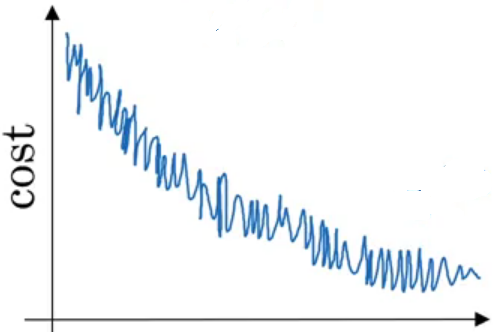Learning rate decay

ItŌĆÖs a technique used to automatically reduce learning rate after each epoch. Decay rate is a hyperparameter.

$$╬▒ = \frac{1}{1+ decayrate + epochnum} . ╬▒_0$$

Momentum

Momentum is a method used to accelerate gradient descent. The idea is to add an extra term to the equation to accelerate descent steps.

$$╬Ė_{j_{t+1}} := ╬Ė_{j_t} – ╬▒ \frac{\partial J(╬Ė_{j_t})}{\partial ╬Ė_j} \color{blue} {+ ╬╗ (╬Ė_{j_t} – ╬Ė_{j_{t-1}})}$$

Below another way to write the expression:

$$v(╬Ė_{j},t) = ╬▒ . \frac{\partial J(╬Ė_j)}{\partial ╬Ė_j} + ╬╗ . v(╬Ė_{j},t-1) \\ ╬Ė_{j} := ╬Ė_{j} – \color{blue} {v(╬Ė_{j},t)}$$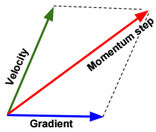Nesterov Momentum is a slightly different version of momentum method.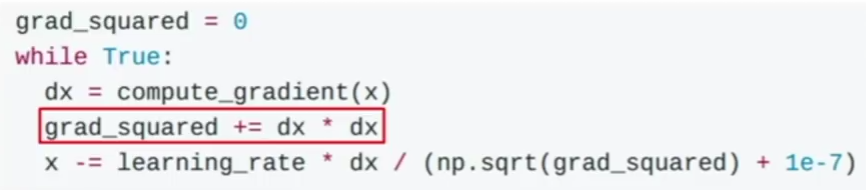The problem in this method is that the term grad_squared becomes large after running many gradient descent steps. The term grad_squared is used to accelerate gradient descent when gradients are small, and slow down gradient descent when gradients are large.

RMSprop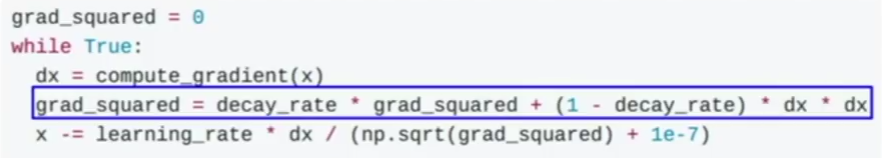The term decay_rate is used to apply exponential smoothing on grad_squared term.

Adam is a combination of Momentum and RMSprop.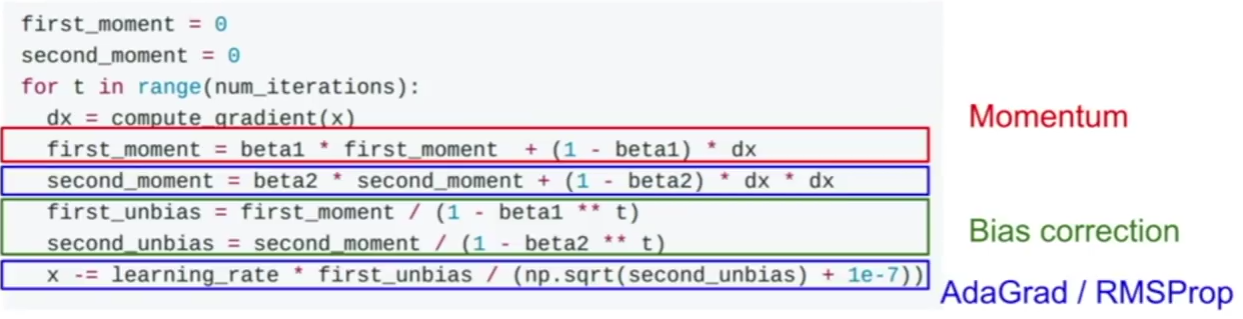Normal equation

To minimize the cost function $$J(╬Ė) = \frac{1}{2} \sum_{i=1}^{n} (y^{(i)}-h_{╬Ė}(x^{(i)}))^{2} = \frac{1}{2} (X╬Ė – y)^T(X╬Ė – y)$$, we need to solve the equation:

$$\frac{\partial J(╬Ė)}{\partial ╬Ė} = 0 \\ \frac{\partial trace(J(╬Ė))}{\partial ╬Ė} = 0 \\ \frac{\partial trace((X╬Ė – y)^T(X╬Ė – y))}{\partial ╬Ė} = 0 \\ \frac{\partial trace(╬Ė^TX^TX╬Ė – ╬Ė^TX^Ty – y^TX╬Ė + y^Ty)}{\partial ╬Ė} = 0$$ $$\frac{\partial trace(╬Ė^TX^TX╬Ė) – trace(╬Ė^TX^Ty) – trace(y^TX╬Ė) + trace(y^Ty))}{\partial ╬Ė} = 0 \\ \frac{\partial trace(╬Ė^TX^TX╬Ė) – trace(╬Ė^TX^Ty) – trace(y^TX╬Ė))}{\partial ╬Ė} = 0$$ $$\frac{\partial trace(╬Ė^TX^TX╬Ė) – trace(y^TX╬Ė) – trace(y^TX╬Ė))}{\partial ╬Ė} = 0 \\ \frac{\partial trace(╬Ė^TX^TX╬Ė) – 2 trace(y^TX╬Ė))}{\partial ╬Ė} = 0 \\ \frac{\partial trace(╬Ė╬Ė^TX^TX)}{\partial ╬Ė} – 2 \frac{\partial trace(╬Ėy^TX))}{\partial ╬Ė} = 0 \\ 2 X^TX╬Ė – 2 X^Ty = 0 \\ X^TX╬Ė= X^Ty \\ ╬Ė = {(X^TX)}^{-1}X^Ty$$

If $$X^TX$$ is singular, then we need to calculate the pseudo inverse instead of the inverse.

Newton method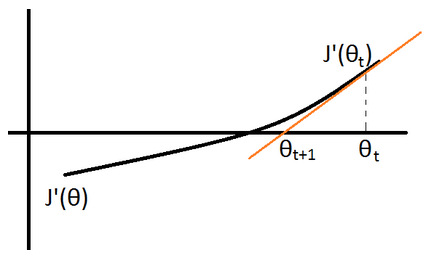$$J”(╬Ė_{t}) := \frac{J'(╬Ė_{t+1}) – J'(╬Ė_{t})}{╬Ė_{t+1} – ╬Ė_{t}}$$ $$\rightarrow ╬Ė_{t+1} := ╬Ė_{t} – \frac{J'(╬Ė_{t})}{J”(╬Ė_{t})}$$

Matrix differentiation

To minimize the cost function: $$J(╬Ė) = \frac{1}{2} \sum_{i=1}^{n} (y^{(i)}-h_{╬Ė}(x^{(i)}))^{2} = \frac{1}{2} (X╬Ė – y)^T(X╬Ė – y)$$, we need to solve the equation:

$$\frac{\partial J(╬Ė)}{\partial ╬Ė} = 0 \\ \frac{\partial ╬Ė^TX^TX╬Ė – ╬Ė^TX^Ty – y^TX╬Ė + y^Ty}{\partial ╬Ė} = 2X^TX╬Ė – \frac{\partial ╬Ė^TX^Ty}{\partial ╬Ė} – X^Ty = 0$$

$$2X^TX╬Ė – \frac{\partial y^TX╬Ė}{\partial ╬Ė} – X^Ty = 2X^TX╬Ė – 2X^Ty = 0$$ (Note: In matrix differentiation: $$\frac{\partial A╬Ė}{\partial ╬Ė} = A^T$$ and $$\frac{\partial ╬Ė^TA╬Ė}{\partial ╬Ė} = 2A^T╬Ė$$)

we can deduce $$X^TX╬Ė = X^Ty$$ and $$╬Ė = (X^TX)^{-1}X^Ty$$

# Logistic Regression

Below a table that shows tumor types by size.

 x = Tumor Size (cm) y = Tumor Type (Benign=0, Malignant=1) 1 0 1 0 2 0 2 1 3 1 3 1

Given x, y is distributed according to the Bernoulli distribution with probability of success p = E[y|x].

$$P(y|x;╬Ė) = p^y (1-p)^{(1-y)}$$

We define h(x) as a function that returns the expected value (p) of the distribution. We will define this function as:

$$E[y|x] = h_{╬Ė}(x) = g(╬Ė^T x) = \frac{1}{1+exp(-╬Ė^T x)}$$. g is called Sigmoid (or logistic) function.

P(y|x; ╬Ė) = $$h_{╬Ė}(x)^y (1-h_{╬Ė}(x))^{(1-y)}$$

We need to find ╬Ė that maximizes this probability for all values of x. In other words, we need to find ╬Ė that maximizes the likelihood function L:

$$L(╬Ė)=P(\overrightarrow{y}|X;╬Ė)=\prod_{i=1}^{m} P(y^{(i)}|x^{(i)};╬Ė)$$

Or maximize the log likelihood function l:

$$l(╬Ė)=log(L(╬Ė)) = \sum_{i=1}^{m} log(P(y^{(i)}|x^{(i)};╬Ė ))$$ $$= \sum_{i=1}^{m} y^{(i)} log(h_{╬Ė}(x^{(i)}))+ (1-y^{(i)}) log(1-h_{╬Ė}(x^{(i)}))$$

Or minimize the $$-l(╬Ė) = \sum_{i=1}^{m} -y^{(i)} log(h_{╬Ė}(x^{(i)})) – (1-y^{(i)}) log(1-h_{╬Ė}(x^{(i)})) = J(╬Ė)$$

J(╬Ė) is convex, to minimize it, we need to solve the equation $$\frac{\partial J(╬Ė)}{\partial ╬Ė} = 0$$.

There are many methods to solve this equation:

$$╬Ė_{j} := ╬Ė_{j} – ╬▒ \sum_{i=1}^{n} (h_{╬Ė}(x^{(i)}) – y^{(i)}) * x_j^{(i)}$$

Logit function (inverse of logistic function)

Logit function is defined as follow: $$logit(p) = log(\frac{p}{1-p})$$

The idea in the use of this function is to transform the interval of p (outcome) from [0,1] to [0, Ōł×]. So instead of applying linear regression on p, we will apply it on logit(p).

Once we find ╬Ė that maximizes the Likelihood function, we can then estimate logit(p) given a value of x ($$logit(p) = h_{╬Ė}(x)$$). p can be then calculated using the following formula:

$$p = \frac{1}{1+exp(-logit(h_{╬Ė}(x)))}$$

# Multinomial Logistic Regression (using maximum likelihood estimation)

In multinomial logistic regression (also called Softmax Regression), y could have more than two outcomes {1,2,3,…,k}.

Below a table that shows tumor types by size.

 x = Tumor Size (cm) y = Tumor Type (Type1= 1, Type2= 2, Type3= 3) 1 1 1 1 2 2 2 2 2 3 3 3 3 3

Given x, we can define a multinomial distribution with probabilities of success $$\phi_j = E[y=j|x]$$.

$$P(y=j|x;\Theta) = ŽĢ_j \\ P(y=k|x;\Theta) = 1 – \sum_{j=1}^{k-1} ŽĢ_j \\ P(y|x;\Theta) = ŽĢ_1^{1\{y=1\}} * … * ŽĢ_{k-1}^{1\{y=k-1\}} * (1 – \sum_{j=1}^{k-1} ŽĢ_j)^{1\{y=k\}}$$

We define $$\tau(y)$$ as a function that returns a $$R^{k-1}$$ vector with value 1 at the index y: $$\tau(y) = \begin{bmatrix}0\\0\\1\\0\\0\end{bmatrix}$$, when $$y \in \{1,2,…,k-1\}$$, .

and $$\tau(y) = \begin{bmatrix}0\\0\\0\\0\\0\end{bmatrix}$$, when y = k.

We define $$\eta(x)$$ as a $$R^{k-1}$$ vector = $$\begin{bmatrix}log(\phi_1/\phi_k)\\log(\phi_2/\phi_k)\\…\\log(\phi_{k-1}/\phi_k)\end{bmatrix}$$

$$P(y|x;\Theta) = 1 * exp(╬Ę(x)^T * \tau(y) – (-log(\phi_k)))$$

This form is an exponential family distribution form.

We can invert $$\eta(x)$$ and find that:

$$ŽĢ_j = ŽĢ_k * exp(╬Ę(x)_j)$$ $$= \frac{1}{1 + \frac{1-ŽĢ_k}{ŽĢ_k}} * exp(╬Ę(x)_j)$$ $$=\frac{exp(╬Ę(x)_j)}{1 + \sum_{c=1}^{k-1} ŽĢ_c/ŽĢ_k}$$ $$=\frac{exp(╬Ę(x)_j)}{1 + \sum_{c=1}^{k-1} exp(╬Ę(x)_c)}$$

If we define ╬Ę(x) as linear function, $$╬Ę(x) = ╬ś^T x = \begin{bmatrix}╬ś_{1,1} x_1 +… + ╬ś_{n,1} x_n \\╬ś_{1,2} x_1 +… + ╬ś_{n,2} x_n\\…\\╬ś_{1,k-1} x_1 +… + ╬ś_{n,k-1} x_n\end{bmatrix}$$, and ╬ś is a $$R^{n*(k-1)}$$ matrix.

Then: $$ŽĢ_j = \frac{exp(╬ś_j^T x)}{1 + \sum_{c=1}^{k-1} exp(╬ś_c^T x)}$$

The hypothesis function could be defined as: $$h_╬ś(x) = \begin{bmatrix}\frac{exp(╬ś_1^T x)}{1 + \sum_{c=1}^{k-1} exp(╬ś_c^T x)} \\…\\ \frac{exp(╬ś_{k-1}^T x)}{1 + \sum_{c=1}^{k-1} exp(╬ś_c^T x)} \end{bmatrix}$$

We need to find ╬ś that maximizes the probabilities P(y=j|x;╬ś) for all values of x. In other words, we need to find ╬Ė that maximizes the likelihood function L:

$$L(╬Ė)=P(\overrightarrow{y}|X;╬Ė)=\prod_{i=1}^{m} P(y^{(i)}|x^{(i)};╬Ė)$$ $$=\prod_{i=1}^{m} \phi_1^{1\{y^{(i)}=1\}} * … * \phi_{k-1}^{1\{y^{(i)}=k-1\}} * (1 – \sum_{j=1}^{k-1} \phi_j)^{1\{y^{(i)}=k\}}$$ $$=\prod_{i=1}^{m} \prod_{c=1}^{k-1} \phi_c^{1\{y^{(i)}=c\}} * (1 – \sum_{j=1}^{k-1} \phi_j)^{1\{y^{(i)}=k\}}$$

$$=\prod_{i=1}^{m} \prod_{c=1}^{k-1} \phi_c^{1\{y^{(i)}=c\}} * (1 – \sum_{j=1}^{k-1} \phi_j)^{1\{y^{(i)}=k\}}$$ and $$ŽĢ_j = \frac{exp(╬ś_j^T x)}{1 + \sum_{c=1}^{k-1} exp(╬ś_c^T x)}$$

# Multinomial Logistic Regression (using cross-entropy minimization)

In this section, we will try to minimize the cross-entropy between Y and estimated $$\widehat{Y}$$.

We define $$W \in R^{d*n}$$, $$b \in R^{d}$$ such as $$S(W x + b) = \widehat{Y}$$, S is the Softmax function, k is the number of outputs, and $$x \in R^n$$.

To estimate W and b, we will need to minimize the cross-entropy between the two probability vectors Y and $$\widehat{Y}$$.

The cross-entropy is defined as below:

$$D(\widehat{Y}, Y) = -\sum_{j=1}^d Y_j Log(\widehat{Y_j})$$

Example:

if $$\widehat{y} = \begin{bmatrix}0.7 \\0.1 \\0.2 \end{bmatrix} \& \ y=\begin{bmatrix}1 \\0 \\0 \end{bmatrix}$$ then $$D(\widehat{Y}, Y) = D(S(W x + b), Y) = -1*log(0.7)$$

We need to minimize the entropy for all training examples, therefore we will need to minimize the average cross-entropy of the entire training set.

$$L(W,b) = \frac{1}{m} \sum_{i=1}^m D(S(W x^{(i)} + b), y^{(i)})$$, L is called the loss function.

If we define $$W = \begin{bmatrix} — ╬Ė_1 — \\ — ╬Ė_2 — \\ .. \\ — ╬Ė_d –\end{bmatrix}$$ such as:

$$╬Ė_1=\begin{bmatrix}╬Ė_{1,0}\\╬Ė_{1,1}\\…\\╬Ė_{1,n}\end{bmatrix}, ╬Ė_2=\begin{bmatrix}╬Ė_{2,0}\\╬Ė_{2,1}\\…\\╬Ė_{2,n}\end{bmatrix}, ╬Ė_d=\begin{bmatrix}╬Ė_{d,0}\\╬Ė_{d,1}\\…\\╬Ė_{d,n}\end{bmatrix}$$

We can then write $$L(W,b) = \frac{1}{m} \sum_{i=1}^m D(S(W x^{(i)} + b), y^{(i)})$$

$$= \frac{1}{m} \sum_{i=1}^m \sum_{j=1}^d 1^{\{y^{(i)}=j\}} log(\frac{exp(╬Ė_k^T x^{(i)})}{\sum_{k=1}^d exp(╬Ė_k^T x^{(i)})})$$

For d=2 (nbr of class=2), $$= \frac{1}{m} \sum_{i=1}^m 1^{\{y^{(i)}=\begin{bmatrix}1 \\ 0\end{bmatrix}\}} log(\frac{exp(╬Ė_1^T x^{(i)})}{exp(╬Ė_1^T x^{(i)}) + exp(╬Ė_2^T x^{(j)})}) + 1^{\{y^{(i)}=\begin{bmatrix}0 \\ 1\end{bmatrix}\}} log(\frac{exp(╬Ė_2^T x^{(i)})}{exp(╬Ė_1^T x^{(i)}) + exp(╬Ė_2^T x^{(i)})})$$

$$1^{\{y^{(i)}=\begin{bmatrix}1 \\ 0\end{bmatrix}\}}$$ means that the value is 1 if $$y^{(i)}=\begin{bmatrix}1 \\ 0\end{bmatrix}$$ otherwise the value is 0.

To estimate $$╬Ė_1,…,╬Ė_d$$, we need to calculate the derivative and update $$╬Ė_j = ╬Ė_j – ╬▒ \frac{\partial L}{\partial ╬Ė_j}$$

# Kernel regression

Kernel regression is a non-linear model. In this model we define the hypothesis as the sum of kernels.

$$\widehat{y}(x) = ŽĢ(x) * ╬Ė = ╬Ė_0 + \sum_{i=1}^d K(x, ╬╝_i, ╬╗) ╬Ė_i$$ such as:

$$ŽĢ(x) = [1, K(x, ╬╝_1, ╬╗),…, K(x, ╬╝_d, ╬╗)]$$ and $$╬Ė = [╬Ė_0, ╬Ė_1,…, ╬Ė_d]$$

For example, we can define the kernel function as : $$K(x, ╬╝_i, ╬╗) = exp(-\frac{1}{╬╗} ||x-╬╝_i||^2)$$

Usually we select d = number of training examples, and $$╬╝_i = x_i$$

Once the vector ŽĢ(X) calculated, we can use it as new engineered vector, and then use the normal equation to find ╬Ė:

$$╬Ė = {(ŽĢ(X)^TŽĢ(X))}^{-1}ŽĢ(X)^Ty$$

# Bayes Point Machine

The Bayes Point Machine is a Bayesian linear classifier that can be converted to a nonlinear classifier by using feature expansions or kernel methods as the Support Vector Machine (SVM).

More details will be provided.

# Ordinal Regression

Ordinal Regression is used for predicting an ordinal variable. An ordinal variable is a categorical variable for which the possible values are ordered (eg. size: Small, Medium, Large).

More details will be provided.

# Poisson Regression

Poisson regression assumes the output variable Y has a Poisson distribution, and assumes the logarithm of its expected value can be modeled by a linear function.

log(E[Y|X]) = log(╬╗) = ╬Ė.x

# Hashing

Feature hashing is used to convert a categorical feature into a small-dimension feature space.

For example a vocabulary of words can be represented in a 5 dimensional space $$\{0,1\}^5$$ (e.g. Apple ŌåÆ [1,0,1,1,0]).

Hashing introduces noise as collisions are permitted (CarŌåÆ [0,0,0,1,1], FlightŌåÆ [0,0,0,1,1]).

# Embedding

With embedding the representation is more dense (e.g. Car ŌåÆ [0.4,0.9,0.1,0,0.1])

# Bucketing

Bucketing is a technique for decomposing feature values into buckets.

# Crossing

Crossing features is combining multiple features in one feature.

# Neural Network

A neural network is a non-linear classifier (separator is not a linear function). It can also be used for regression.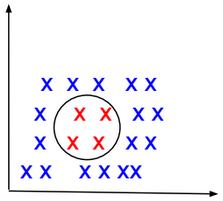A Shallow neural network is a one hidden layer neural network.

A Vanilla neural network is a regular neural network having layers that do not form cycles.

TensorFlow Playground is an interactive web interface for learning neural networks: http://playground.tensorflow.org.

# Computational Graph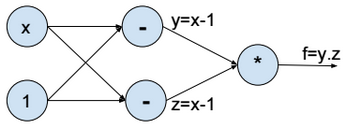Above the computational graph for the function $$f(x) = (x-1)^2$$.

Forward propagation

To minimize the function f, we assign a random value to x (e.g. x = 2), then we evaluate y, z, and f (forward propagation).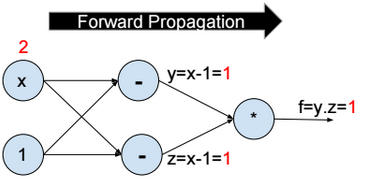Backward propagation

Then we compute the partial derivative of f with respect to x step by step (Backward propagation).

$$\frac{\partial f}{\partial x} = \frac{\partial f}{\partial y}*\frac{\partial y}{\partial x} + \frac{\partial f}{\partial z}*\frac{\partial z}{\partial x} = 2 \\ \frac{\partial f}{\partial y} = z = 1 \\ \frac{\partial f}{\partial z} = y = 1 \\ \frac{\partial y}{\partial x} = \frac{\partial z}{\partial x} = 1$$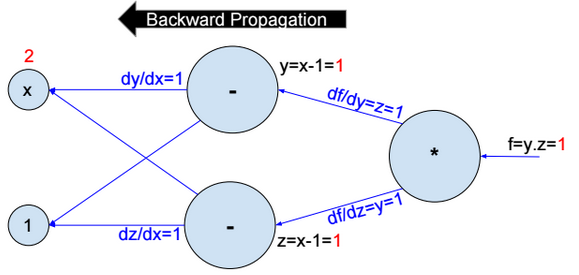Then we update $$x:= x – ?.\frac{\partial f}{\partial x}$$.

We repeat the operation until convergence.

# Activation functions

Activation functions introduce nonlinearity into models. The most used activation functions are:

Sigmoid

$$f(x) = \frac{1}{1+exp(-x)}$$

Sigmoid has a positive and non-zero centred output (sigmoid(0) ? 0.5).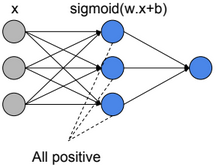When all activation units are positive, then weight update will be in the same direction (all positive or all negative updates) and that will cause a zigzag path during optimization.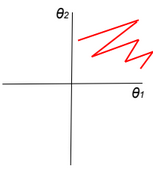$$z=?w_i.a_i+b \\ \frac{dL}{dw_i}=\frac{dL}{dz}.\frac{dz}{dw_i}=\frac{dL}{dz}.ai$$

If all ai>0, then the gradient will have the same sign as $$\frac{dL}{dz}$$ (all positive or all negative).

TanH

$$f(x) = \frac{2}{1+exp(-2x)} -1$$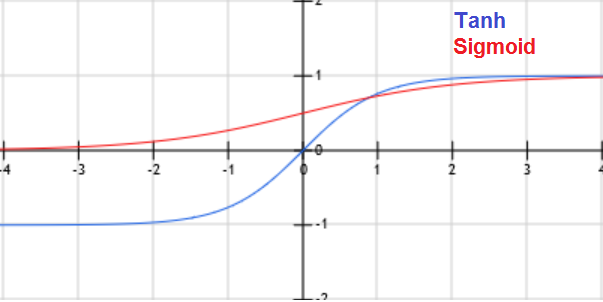When x is large, the derivative of the sigmoid or Tanh function is around zero (vanishing gradient/saturation).

ReLU (Rectified Linear Unit)

f(x) = max(0, x)

Leaky ReLU

f(x) = max(0.01x, x)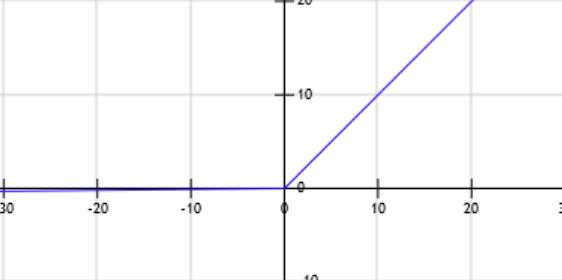Leaky Relu was introduced to fix the ōDying Reluö problem.

$$z=?w_i.a_i+b \\ f=Relu(z) \\ \frac{dL}{dw_i}=\frac{dL}{df}.\frac{df}{dz}.\frac{dz}{dw_i}$$

When z becomes negative, then the derivative of f becomes equal to zero, and the weights stop being updated.

PRelu (Parametric Rectifier)

f(x) = max(?.x, x)

ELU (Exponential Linear Unit)

f(x) = {x if x>0 otherwise ?.(exp(x)-1)}

Other activation functions: Maxout

# Cost function

$$J(?) = \frac{1}{m} \sum_{i=1}^{m} loss(y^{(i)}, f(x^{(i)}; ?))$$

We need to find ? that minimizes the cost function: $$\underset{?}{argmin}\ J(?)$$

# Neural Network Regression

Neural Network regression has no activation function at the output layer.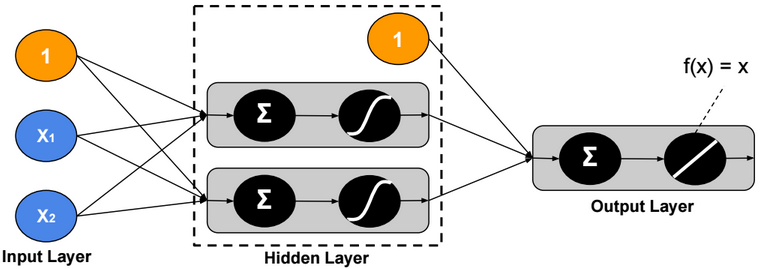L1 Loss function

$$loss(y,?) = |y – ?|$$

L2 Loss function

$$loss(y,?) = (y – ?)^2$$

Hinge loss function

Hinge loss function is recommended when there are some outliers in the data.

$$loss(y,?) = max(0, |y-?| – m)$$

# Two-Class Neural Network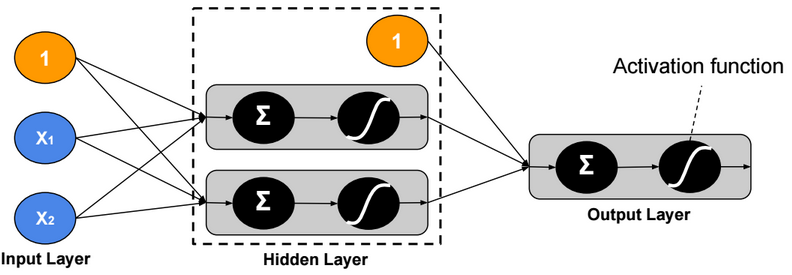Binary Cross Entropy Loss function

$$loss(y,?) = – y.log(?) – (1-y).log(1 – ?)$$

# Multi-Class Neural Network – One-Task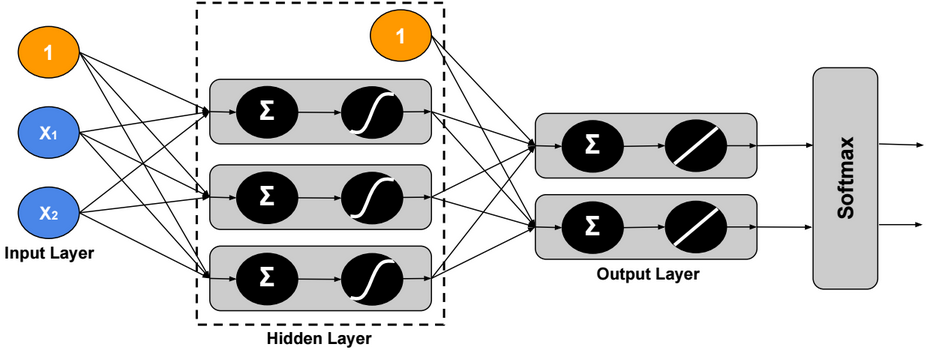Using Softmax, the output ? is modeled as a probability distribution, therefore we can assign only one label to each example.

Cross Entropy Loss function

$$loss(Y,\widehat{Y}) = -\sum_{j=1}^c Y_{j}.log(\widehat{Y}_{j})$$

Hinge Loss (SVM) function

$$y = \begin{bmatrix} 1 \\ 0 \\ 0 \end{bmatrix},\ ? = \begin{bmatrix} 2 \\ -5 \\ 3 \end{bmatrix} \\ loss(y,?) = \sum_{c?1} max(0, ?_c – ?_1 + m)$$

For m = 1, the sum will be equal to 2.

# Multi-Class Neural Network – Multi-Task

In this version, we assign multiple labels to each example.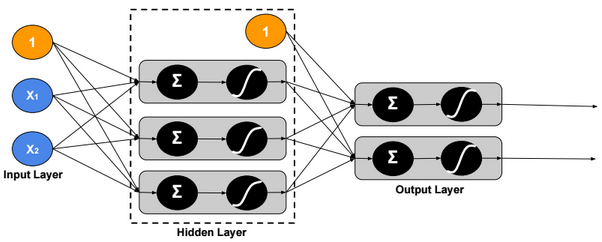Loss function

$$loss(Y,\widehat{Y}) = \sum_{j=1}^c – Y_j.log(\widehat{Y}_j) – (1-Y_j).log(1 – \widehat{Y}_j)$$

# Regularization

Regularization is a very important technique to prevent overfitting.

DropoutFor each training example, ignore randomly p activation nodes of each hidden layer. p is called dropout rate (p?[0,1]). When testing, scale activations by the dropout rate p.

Inverted Dropout

With inverted dropout, scaling is applied at the training time, but inversely. First, dropout all activations by dropout factor p, and second, scale them by inverse dropout factor 1/p. Nothing needs to be applied at test time.

Data Augmentation

As a regularization technique, we can apply random transformations on input images when training a model.

Early stopping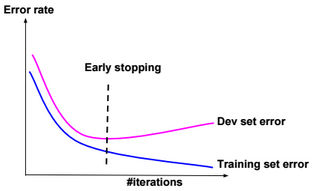Stop when error rates decreases on training data while it increases on dev (cross-validation) data.

L1 regularization

$$J(?) = \frac{1}{m} \sum_{i=1}^{m} loss(y^{(i)}, f(x^{(i)}; ?)) \color{blue} { + ? .\sum_{j} |?_j|}$$

? is called regularization parameter

L2 regularization

$$J(?) = \frac{1}{m} \sum_{i=1}^{m} loss(y^{(i)}, f(x^{(i)}; ?)) \color{blue} { + ? .\sum_{j} ?_j^2}$$

Lp regularization

$$J(?) = \frac{1}{m} \sum_{i=1}^{m} loss(y^{(i)}, f(x^{(i)}; ?)) \color{blue} { + ? .\sum_{j} ?_j^p}$$

For example, if the cost function $$J(?)=(?_1 – 1)^2 + (?_2 – 1)^2$$, then the $$L_2$$ regularized cost function is $$J(?)=(?_1 – 1)^2 + (?_2 – 1)^2 + ? (?_1^2 + ?_2^2)$$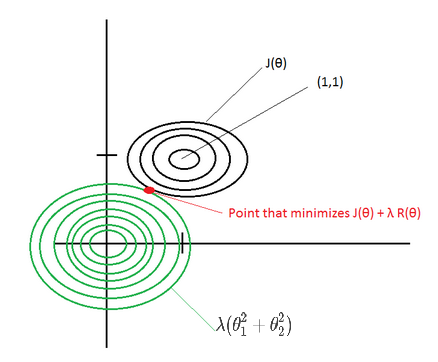If ? is large, then the point that minimizes the regularized J(?) will be around (0,0) –> Underfitting.

If ? ~ 0, then the point that minimizes the regularized J(?) will be around (1,1) –> Overfitting.

Elastic net

Combination of L1 and L2 regularizations.

# Normalization

Gradient descent converges quickly when data is normalized Xi ? [-1,1]. If features have different scales, then the update of parameters will not be in the same scale (zig-zag).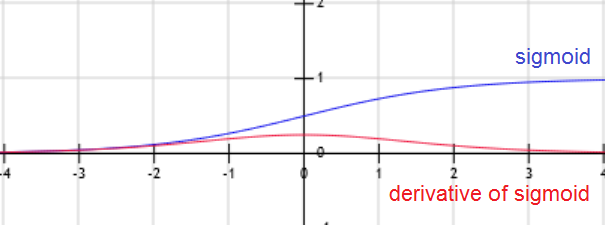For example, if the activation function g is the sigmoid function, then when W.x+b is large g(W.x+b) is around 1, but the derivative of the sigmoid function is around zero. For this reason the gradient converges slowly when the W.x+b is large.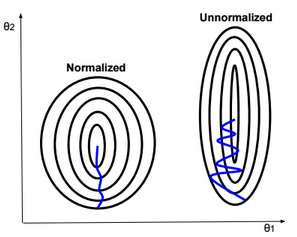Below some normalization functions.

ZScore

$$X:= \frac{X – ?}{?}$$

MinMax

$$X:= \frac{X – min}{max-min}$$

Logistic

$$X:= \frac{1}{1+exp(-X)}$$

LogNormal

$$X:= \frac{1}{?\sqrt{2?}} \int_{0}^{X} \frac{exp(\frac{-(ln(t) – ?)^2}{2?^2})}{t} dt$$

Tanh

$$X:= tanh(X)$$

# Weight Initialization

Weight initialization is important because if weights are too big then activations explode. If weights are too small then gradients will be around zero (no learning).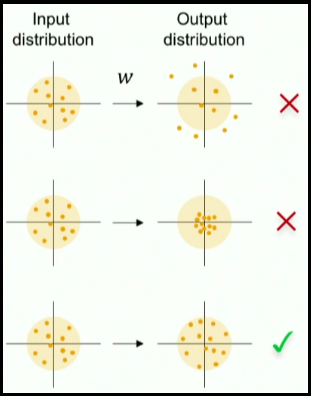When we normalize input data, we make the mean of the input features equals to zero, and the variance equals to one. To keep the activation units normalized too, we can initialize the weights $$W^{(1)}$$ so $$Var(g(W_{j}^{(1)}.x+b_{j}^{(1)}))$$ is equals to one.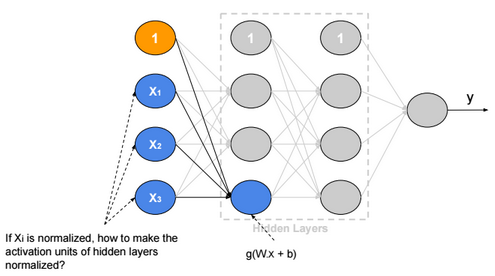If we suppose that g is Relu and $$W_{i,j}, b_j, x_i$$ are independent, then:

$$Var(g(W_{j}^{(1)}.x+b_{j}^{(1)})) = Var(\sum_{i} W_{i,j}^{(1)}.x_i+b_{j}^{(1)}) =\sum_{i} Var(W_{i,j}^{(1)}.x_i) + 0 \\ = \sum_{i} E(x_i)^2.Var(W_{i,j}^{(1)}) + E(W_{i,j}^{(1)})^2.Var(x_i) + Var(W_{i,j}^{(1)}).Var(x_i) \\ = \sum_{i} E(x_i)^2.Var(W_{i,j}^{(1)}) + E(W_{i,j}^{(1)})^2.Var(x_i) + Var(W_{i,j}^{(1)}).Var(x_i) \\ = \sum_{i} 0 + 0 + Var(W_{i,j}^{(1)}).Var(x_i) = n.Var(W_{i,j}^{(1)}).Var(x_i)$$

Xavier initialization

If we define $$W_{i,j}^{(1)} ? N(0,\frac{1}{\sqrt{n}})$$, then the initial variance of activation units will be one (n is number of input units).

We can apply this rule on all weights of the neural network.

# Batch Normalization

Batch normalization is a technique to provide any layer in a Neural Network with normalized inputs. Batch Normalization has a regularizing effect.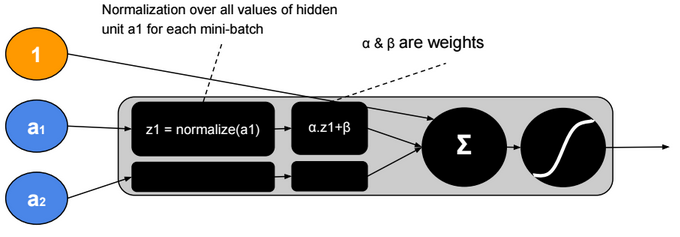After training, ? will converge to the standard deviation of the mini-batches and ? will converge to the mean. The ?, ? parameters give more flexibility when shifting or scaling is needed.

# Hyperparameters

Neural network hyperparameters are:

• Learning rate (?) (e.g. 0.1, 0.01, 0.001,…)
• Number of hidden units
• Number of layers
• Mini-bach size
• Momentum rate (e.g. 0.9)
• Adam optimization parameters (e.g. ?1=0.9, ?2=0.999, ?=0.00000001)
• Learning rate decay

# Local Minimum

The probability that gradient descent gets stuck in a local minimum in a high dimensional space is extremely low. We could have a saddle point, but itÆs rare to have a local minimum.

# Transfer Learning

Transfer Learning consists in the use of parameters of a trained model when training new hidden layers of an extended version of that model.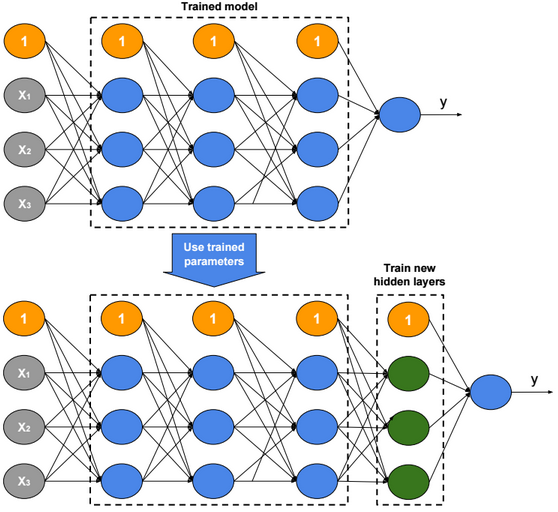# Characteristics of Machine Learning Algorithms

Discriminative / Generative algorithms

Many machine learning algorithms are based on probabilistic models. Discriminative algorithms learn the conditional probability distribution of y given x p(y|x). Generative algorithms learn the joint probability distribution p(y,x) = p(y|x) * p(x), and therefore take in consideration the distribution of x.

Parametric & Non-Parametric algorithms

Neural Network, SVM,… are non-parametric algorithms because they have no fixed parameter vector; depending on the richness of the data we can choose the size of the parameter vector that we need (ex. we can add hidden units, change SVM kernels,…)

Capacity

The capacity is controlled by parameters called Hyperparameters (ex. degree p of a polynomial predictor, kernel choice in SVM, number of layers in a neural network).

# Summary of Machine Learning Algorithms

The table below describes briefly each machine learning algorithm.

 Algorithm Description Characteristics Linear regression To use when Y is normally-distributed Discriminative Parametric Logistic regression To use when Y is Bernoulli-distributed Discriminative Parametric Multinomial logistic regression (softmax regression) To use when Y is multinomially-distributed There are two versions of the algorithm, one based on maximum likelihood maximization, and one based on cross-entropy minimization. Discriminative Parametric Gaussian Discriminant Analysis Supervised classification algorithm To use when Y is Bernoulli distributed and the conditional distribution of X given Y is multivariate Gaussian Generative Parametric Naive Bayes Algorithm Supervised classification algorithm To use when Y is Bernoulli, and the conditional distribution of X given Y is Bernoulli and X features are conditionally independent Generative Parametric EM Unsupervised soft-clustering algorithm Generative Parametric Principal Component Analysis Reduce the dimensionality of X. Calculate eigenvectors for $$XX^T$$. Use eigenvectors with higher eigenvalues to transform data $$x^{(i)} := (u_1^T x^{(i)}, u_2^T x^{(i)},…, , u_k^T x^{(i)})$$ Factor Analysis Reduce the dimensionality of X To use when X is Gaussian. Transform X to Z matrix with lower dimensionality (x ? ?+?z). Neural Network Non-linear classifier Discriminative Non-Parametric K-Nearest Neighbor Regression Predict y as the average of values y1,y2,ģ,yk of k nearest neighbors of x Discriminative Non-Parametric K-Nearest Neighbor Classifier Predict y as the most common class among the k nearest neighbors of x Discriminative Non-Parametric Support Vector Machine Find a separator that maximizes the margin between two classes Discriminative Non-Parametric K-means Unsupervised hard-clustering algorithm Discriminative Non-Parametric